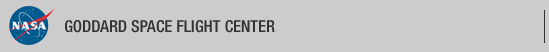+ Visit NASA.gov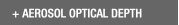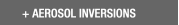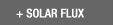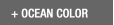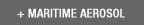Web Site Feature AERONET Data Synergy Tool - Access Earth Science data sets for AERONET sites+Home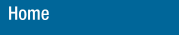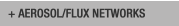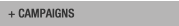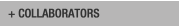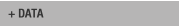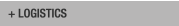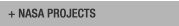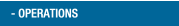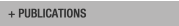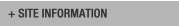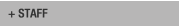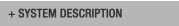AERONET DATA ACCESS

AERONET Site Lists (V3)

+ All Lists# Report of DCP transmissions

 Status Legend

AERONET No 6 [1317E33A] - 28 msg rcvd (12531 b) site : GSFC (Last Tx is before schedule by 0 min 3 sec
28 messages stepped outside their assigned time window)
AERONET No 26 [1316E1C0] - 28 msg rcvd (17383 b) site : GSFC (Last Tx is late by 0 min 1 sec)
AERONET No 28 [13101F58] - 28 msg rcvd (13301 b) site : White_Sands_HELSTF (Last Tx is precisely on time)
AERONET No 59 [131A03AE] - 28 msg rcvd (4157 b) site : Sevilleta (Last Tx is precisely on time)
AERONET No 134  - 28 msg rcvd (17330 b) site : GSFC (Last Tx is before schedule by 0 min 3 sec
28 messages stepped outside their assigned time window , 1b)
AERONET No 163  - 28 msg rcvd (12979 b) site : UCSB (Last Tx is precisely on time)
AERONET No 197 [1319A458] - 28 msg rcvd (3447 b) site : Rio_Branco (Last Tx is precisely on time)
AERONET No 306 [131A40A4] - 28 msg rcvd (6176 b) site : Easton-MDE (Last Tx is precisely on time , 35b)
AERONET No 307 [1318152C] - 28 msg rcvd (14131 b) site : Ames (Last Tx is late by 0 min 1 sec , 5b)
AERONET No 317 [131982B4] - 28 msg rcvd (14662 b) site : Tudor_Hill (Last Tx is precisely on time)
AERONET No 319 [131820B6] - 28 msg rcvd (8063 b) site : Trelew (Last Tx is late by 0 min 1 sec 284s)
AERONET No 330 [131481D2] - 28 msg rcvd (22978 b) site : GSFC (Last Tx is late by 0 min 1 sec)
AERONET No 365 [131A53D2] - 28 msg rcvd (15037 b) site : Ji_Parana_SE (Last Tx is precisely on time , 16b)
AERONET No 374 [131713BE] - 28 msg rcvd (7299 b) site : TABLE_MOUNTAIN_CA (Last Tx is precisely on time)
AERONET No 409 [131AA356] - 28 msg rcvd (5171 b) site : Mingo (Last Tx is precisely on time)
AERONET No 601 [1319E752] - 27 msg rcvd (3024 b) site : CCNY ()
AERONET No 624 [131AE05C] - 28 msg rcvd (3108 b) site : CUIABA-MIRANDA ()
AERONET No 635 [1319D2C8] - 28 msg rcvd (23977 b) site : Alta_Floresta (Last Tx is precisely on time)
AERONET No 707 [1316D45A] - 28 msg rcvd (2108 b) site : Ascension_Island (Last Tx is late by 0 min 1 sec)

# Aeronet No 6 [1317E33A] (GSFC)

Last Tx is before schedule by 0 min 3 sec
28 messages stepped outside their assigned time window

08:12:2019,10:41:47 (UTC) 'G' (DCP total: 87) Cimel : 6 time corr. = -23 sec (DCP Volt = 13.00 Lat= 38.993 Long= -76.840(0.0 km from GSFC))
08:12:2019,11:41:47 (UTC) 'G' (DCP total: 87) Cimel : 6 time corr. = -23 sec (DCP Volt = 13.00 Lat= 38.993 Long= -76.840(0.0 km from GSFC))
08:12:2019,12:41:47 (UTC) 'G' (DCP total: 87) Cimel : 6 time corr. = -23 sec (DCP Volt = 13.00 Lat= 38.993 Long= -76.840(0.1 km from GSFC))
08:12:2019,13:41:47 (UTC) 'G' (DCP total: 786) Cimel : 6 time corr. = -23 sec (DCP Volt = 13.00 Lat= 38.993 Long= -76.840(0.0 km from GSFC)) staD nsu staD nsu staD nsu staD nsu staD nsu staD nsu
08:12:2019,22:41:47 (UTC) 'G' (DCP total: 777) Cimel : 6 time corr. = -23 sec (DCP Volt = 13.00 Lat= 38.993 Long= -76.840(0.0 km from GSFC)) nsu staD nsu staD nsu staD nsu staD nsu staD nsu staD
08:12:2019,23:41:47 (UTC) 'G' (DCP total: 87) Cimel : 6 time corr. = -23 sec (DCP Volt = 13.00 Lat= 38.993 Long= -76.840(0.0 km from GSFC))
09:12:2019,00:41:47 (UTC) 'G' (DCP total: 87) Cimel : 6 time corr. = -23 sec (DCP Volt = 13.00 Lat= 38.992 Long= -76.840(0.0 km from GSFC))
09:12:2019,01:41:47 (UTC) 'G' (DCP total: 87) Cimel : 6 time corr. = -24 sec (DCP Volt = 13.00 Lat= 38.992 Long= -76.840(0.0 km from GSFC))
09:12:2019,02:41:47 (UTC) 'G' (DCP total: 87) Cimel : 6 time corr. = -23 sec (DCP Volt = 13.00 Lat= 38.993 Long= -76.840(0.0 km from GSFC))
09:12:2019,03:41:47 (UTC) 'G' (DCP total: 87) Cimel : 6 time corr. = -23 sec (DCP Volt = 13.00 Lat= 38.993 Long= -76.840(0.0 km from GSFC))
09:12:2019,04:41:47 (UTC) 'G' (DCP total: 87) Cimel : 6 time corr. = -24 sec (DCP Volt = 13.00 Lat= 38.993 Long= -76.840(0.0 km from GSFC))
09:12:2019,05:41:47 (UTC) 'G' (DCP total: 87) Cimel : 6 time corr. = -23 sec (DCP Volt = 13.00 Lat= 38.993 Long= -76.840(0.0 km from GSFC))
09:12:2019,06:41:47 (UTC) 'G' (DCP total: 87) Cimel : 6 time corr. = -23 sec (DCP Volt = 13.00 Lat= 38.993 Long= -76.840(0.0 km from GSFC))
09:12:2019,07:41:47 (UTC) 'G' (DCP total: 87) Cimel : 6 time corr. = -24 sec (DCP Volt = 13.00 Lat= 38.993 Long= -76.840(0.0 km from GSFC))
09:12:2019,08:41:47 (UTC) 'G' (DCP total: 87) Cimel : 6 time corr. = -23 sec (DCP Volt = 13.00 Lat= 38.992 Long= -76.840(0.0 km from GSFC))
09:12:2019,09:41:47 (UTC) 'G' (DCP total: 87) Cimel : 6 time corr. = -23 sec (DCP Volt = 13.00 Lat= 38.992 Long= -76.840(0.0 km from GSFC))
09:12:2019,10:41:47 (UTC) 'G' (DCP total: 87) Cimel : 6 time corr. = -24 sec (DCP Volt = 13.00 Lat= 38.993 Long= -76.840(0.1 km from GSFC))
09:12:2019,11:41:47 (UTC) 'G' (DCP total: 87) Cimel : 6 time corr. = -23 sec (DCP Volt = 13.00 Lat= 38.993 Long= -76.840(0.1 km from GSFC))
09:12:2019,12:41:47 (UTC) 'G' (DCP total: 87) Cimel : 6 time corr. = -23 sec (DCP Volt = 13.00 Lat= 38.993 Long= -76.840(0.0 km from GSFC))
09:12:2019,13:41:47 (UTC) 'G' (DCP total: 234) Cimel : 6 time corr. = -24 sec (DCP Volt = 13.00 Lat= 38.993 Long= -76.840(0.0 km from GSFC)) nsu stah stah stah stah stah stah

# Aeronet No 26 [1316E1C0] (GSFC)

Last Tx is late by 0 min 1 sec

08:12:2019,10:32:41 (UTC) 'G' (DCP total: 89) Cimel : 26 time corr. = 0 sec (DCP Volt = 12.80 Lat= 38.993 Long= -76.840(0.0 km from GSFC))
08:12:2019,11:32:41 (UTC) 'G' (DCP total: 89) Cimel : 26 time corr. = 0 sec (DCP Volt = 12.80 Lat= 38.993 Long= -76.840(0.0 km from GSFC))
08:12:2019,12:32:41 (UTC) 'G' (DCP total: 89) Cimel : 26 time corr. = 0 sec (DCP Volt = 12.70 Lat= 38.993 Long= -76.840(0.0 km from GSFC))
08:12:2019,13:32:41 (UTC) 'G' (DCP total: 605) Cimel : 26 time corr. = 0 sec (DCP Volt = 12.80 Lat= 38.993 Long= -76.840(0.0 km from GSFC)) staA ssk staA ssk staA ssk staA
08:12:2019,14:32:41 (UTC) 'G' (DCP total: 1216) Cimel : 26 time corr. = 0 sec (DCP Volt = 14.30 Lat= 38.993 Long= -76.840(0.0 km from GSFC)) ssk staA ssk staA ssk staB ssk all(1) alr(1) all(2) alr(2) all(3) alr(3) all(4)
08:12:2019,15:32:41 (UTC) 'G' (DCP total: 1214) Cimel : 26 time corr. = -1 sec (DCP Volt = 14.10 Lat= 38.993 Long= -76.840(0.0 km from GSFC)) alr(4) blk staA ssk staF nsu all(1) alr(1) all(2) alr(2) all(3) alr(3) all(4) alr(4) nsu
08:12:2019,16:32:41 (UTC) 'G' (DCP total: 1213) Cimel : 26 time corr. = -1 sec (DCP Volt = 14.00 Lat= 38.992 Long= -76.840(0.0 km from GSFC)) pp1(1) pp1(2) pp1(3) pp1(4) blk staD nsu staD nsu staD nsu staF nsu all(1) alr(1)
08:12:2019,17:32:41 (UTC) 'G' (DCP total: 1213) Cimel : 26 time corr. = -2 sec (DCP Volt = 13.70 Lat= 38.993 Long= -76.840(0.0 km from GSFC)) all(2) alr(2) all(3) alr(3) all(4) alr(4) nsu pp1(1) pp1(2) pp1(3) pp1(4) blk staD
08:12:2019,18:32:41 (UTC) 'G' (DCP total: 1216) Cimel : 26 time corr. = -1 sec (DCP Volt = 13.60 Lat= 38.993 Long= -76.840(0.0 km from GSFC)) nsu staD nsu staD nsu staF nsu all(1) alr(1) all(2) alr(2) all(3) alr(3) all(4) alr(4)
08:12:2019,19:32:41 (UTC) 'G' (DCP total: 1214) Cimel : 26 time corr. = -1 sec (DCP Volt = 13.50 Lat= 38.993 Long= -76.840(0.0 km from GSFC)) nsu pp1(1) pp1(2) pp1(3) pp1(4) blk staD nsu staD nsu staD nsu staF nsu all(1) alr(1)
08:12:2019,20:32:41 (UTC) 'G' (DCP total: 1213) Cimel : 26 time corr. = -2 sec (DCP Volt = 13.40 Lat= 38.993 Long= -76.840(0.0 km from GSFC)) all(2) alr(2) all(3) alr(3) all(4) alr(4) nsu pp1(1) pp1(2) pp1(3) pp1(4) blk staD
08:12:2019,21:32:41 (UTC) 'G' (DCP total: 1216) Cimel : 26 time corr. = -1 sec (DCP Volt = 13.30 Lat= 38.992 Long= -76.840(0.0 km from GSFC)) nsu staD nsu staD nsu staF nsu all(1) alr(1) all(2) alr(2) all(3) alr(3) all(4) alr(4)
08:12:2019,22:32:41 (UTC) 'G' (DCP total: 1213) Cimel : 26 time corr. = -1 sec (DCP Volt = 13.10 Lat= 38.993 Long= -76.840(0.0 km from GSFC)) nsu pp1(1) pp1(2) pp1(3) pp1(4) blk staD nsu staD nsu staD nsu staF nsu all(1)
08:12:2019,23:32:41 (UTC) 'G' (DCP total: 1215) Cimel : 26 time corr. = -2 sec (DCP Volt = 13.10 Lat= 38.993 Long= -76.840(0.0 km from GSFC)) alr(1) all(2) alr(2) all(3) alr(3) all(4) alr(4) nsu pp1(1) pp1(2) pp1(3)
09:12:2019,00:32:41 (UTC) 'G' (DCP total: 1215) Cimel : 26 time corr. = -1 sec (DCP Volt = 13.00 Lat= 38.993 Long= -76.840(0.0 km from GSFC)) pp1(4) blk staD nsu staD nsu staD nsu staF nsu all(1) alr(1) all(2) alr(2) all(3) alr(3) all(4)
09:12:2019,01:32:41 (UTC) 'G' (DCP total: 1216) Cimel : 26 time corr. = -1 sec (DCP Volt = 13.00 Lat= 38.993 Long= -76.840(0.0 km from GSFC)) alr(4) nsu pp1(1) pp1(2) pp1(3) pp1(4) blk staA ssk staB ssk all(1) alr(1)
09:12:2019,02:32:41 (UTC) 'G' (DCP total: 1213) Cimel : 26 time corr. = -2 sec (DCP Volt = 13.00 Lat= 38.993 Long= -76.840(0.0 km from GSFC)) all(2) alr(2) all(3) alr(3) all(4) alr(4) blk staA ssk staA ssk staA ssk staA ssk staA
09:12:2019,03:32:41 (UTC) 'G' (DCP total: 254) Cimel : 26 time corr. = -1 sec (DCP Volt = 13.00 Lat= 38.993 Long= -76.840(0.0 km from GSFC)) ssk staA
09:12:2019,04:32:41 (UTC) 'G' (DCP total: 89) Cimel : 26 time corr. = -1 sec (DCP Volt = 13.00 Lat= 38.993 Long= -76.840(0.0 km from GSFC))
09:12:2019,05:32:41 (UTC) 'G' (DCP total: 89) Cimel : 26 time corr. = -2 sec (DCP Volt = 13.00 Lat= 38.993 Long= -76.840(0.0 km from GSFC))
09:12:2019,06:32:41 (UTC) 'G' (DCP total: 89) Cimel : 26 time corr. = -1 sec (DCP Volt = 12.90 Lat= 38.993 Long= -76.840(0.0 km from GSFC))
09:12:2019,07:32:41 (UTC) 'G' (DCP total: 89) Cimel : 26 time corr. = -1 sec (DCP Volt = 12.90 Lat= 38.993 Long= -76.840(0.0 km from GSFC))
09:12:2019,08:32:41 (UTC) 'G' (DCP total: 89) Cimel : 26 time corr. = -2 sec (DCP Volt = 12.90 Lat= 38.993 Long= -76.840(0.0 km from GSFC))
09:12:2019,09:32:41 (UTC) 'G' (DCP total: 89) Cimel : 26 time corr. = -1 sec (DCP Volt = 12.80 Lat= 38.993 Long= -76.840(0.0 km from GSFC))
09:12:2019,10:32:41 (UTC) 'G' (DCP total: 89) Cimel : 26 time corr. = -1 sec (DCP Volt = 12.80 Lat= 38.993 Long= -76.840(0.0 km from GSFC))
09:12:2019,11:32:41 (UTC) 'G' (DCP total: 89) Cimel : 26 time corr. = -2 sec (DCP Volt = 12.80 Lat= 38.992 Long= -76.840(0.0 km from GSFC))
09:12:2019,12:32:41 (UTC) 'G' (DCP total: 89) Cimel : 26 time corr. = -1 sec (DCP Volt = 12.80 Lat= 38.993 Long= -76.840(0.0 km from GSFC))
09:12:2019,13:32:41 (UTC) 'G' (DCP total: 173) Cimel : 26 time corr. = -1 sec (DCP Volt = 12.80 Lat= 38.993 Long= -76.840(0.0 km from GSFC)) ssk stah stah stah

# Aeronet No 28 [13101F58] (White_Sands_HELSTF)

Last Tx is precisely on time

08:12:2019,10:12:21 (UTC) 'G' (DCP total: 88) Cimel : 28 time corr. = 1 sec (DCP Volt = 12.40 Lat= 32.635 Long= -106.339(0.1 km from White_Sands_HELSTF))
08:12:2019,11:12:21 (UTC) 'G' (DCP total: 88) Cimel : 28 time corr. = 0 sec (DCP Volt = 12.40 Lat= 32.635 Long= -106.339(0.1 km from White_Sands_HELSTF))
08:12:2019,12:12:21 (UTC) 'G' (DCP total: 88) Cimel : 28 time corr. = 0 sec (DCP Volt = 12.40 Lat= 32.635 Long= -106.339(0.1 km from White_Sands_HELSTF))
08:12:2019,13:12:21 (UTC) 'G' (DCP total: 88) Cimel : 28 time corr. = 1 sec (DCP Volt = 12.40 Lat= 32.635 Long= -106.339(0.1 km from White_Sands_HELSTF))
08:12:2019,14:12:21 (UTC) 'G' (DCP total: 88) Cimel : 28 time corr. = 0 sec (DCP Volt = 12.40 Lat= 32.635 Long= -106.339(0.1 km from White_Sands_HELSTF))
08:12:2019,15:12:21 (UTC) 'G' (DCP total: 573) Cimel : 28 time corr. = 0 sec (DCP Volt = 12.40 Lat= 32.635 Long= -106.339(0.1 km from White_Sands_HELSTF)) staA nsu staA nsu staA nsu staA nsu staA
08:12:2019,16:12:21 (UTC) 'G' (DCP total: 1212) Cimel : 28 time corr. = 1 sec (DCP Volt = 12.40 Lat= 32.635 Long= -106.339(0.1 km from White_Sands_HELSTF)) nsu staA nsu staB nsu staA nsu staA nsu staA nsu staA nsu staC nsu all(1) alr(1) all(2) alr(2)
08:12:2019,17:12:21 (UTC) 'G' (DCP total: 1214) Cimel : 28 time corr. = 0 sec (DCP Volt = 13.30 Lat= 32.635 Long= -106.339(0.1 km from White_Sands_HELSTF)) all(3) alr(3) all(4) alr(4) nsu pp1(1) pp1(2) pp1(3) pp1(4) staF nsu all(1)
08:12:2019,18:12:21 (UTC) 'G' (DCP total: 1214) Cimel : 28 time corr. = 0 sec (DCP Volt = 13.00 Lat= 32.635 Long= -106.339(0.1 km from White_Sands_HELSTF)) alr(1) all(2) alr(2) all(3) alr(3) all(4) alr(4) nsu pp1(1) pp1(2) pp1(3)
08:12:2019,19:12:21 (UTC) 'G' (DCP total: 1212) Cimel : 28 time corr. = 1 sec (DCP Volt = 12.90 Lat= 32.635 Long= -106.339(0.1 km from White_Sands_HELSTF)) pp1(4) blk staD nsu staD nsu staD nsu staF nsu staD nsu staD nsu staD nsu staF nsu staD nsu staD nsu staD nsu staF
08:12:2019,20:12:21 (UTC) 'G' (DCP total: 536) Cimel : 28 time corr. = 0 sec (DCP Volt = 12.90 Lat= 32.635 Long= -106.339(0.1 km from White_Sands_HELSTF)) nsu staD nsu staD nsu staD nsu staF
08:12:2019,21:12:21 (UTC) 'G' (DCP total: 1212) Cimel : 28 time corr. = 0 sec (DCP Volt = 13.40 Lat= 32.635 Long= -106.339(0.1 km from White_Sands_HELSTF)) nsu staD nsu staD nsu staD nsu staF nsu all(1) alr(1) all(2) alr(2) all(3) alr(3) all(4)
08:12:2019,22:12:21 (UTC) 'G' (DCP total: 1214) Cimel : 28 time corr. = 1 sec (DCP Volt = 13.40 Lat= 32.635 Long= -106.339(0.1 km from White_Sands_HELSTF)) alr(4) nsu pp1(1) pp1(2) pp1(3) pp1(4) blk staD nsu staD nsu staD nsu staF nsu all(1)
08:12:2019,23:12:21 (UTC) 'G' (DCP total: 1215) Cimel : 28 time corr. = 0 sec (DCP Volt = 13.30 Lat= 32.635 Long= -106.339(0.1 km from White_Sands_HELSTF)) alr(1) all(2) alr(2) all(3) alr(3) all(4) alr(4) nsu pp1(1) pp1(2) pp1(3)
09:12:2019,00:12:21 (UTC) 'G' (DCP total: 1213) Cimel : 28 time corr. = 0 sec (DCP Volt = 12.80 Lat= 32.635 Long= -106.339(0.1 km from White_Sands_HELSTF)) pp1(4) blk staC nsu all(1) alr(1) all(2) alr(2) all(3) alr(3) all(4) alr(4) nsu pp1(1)
09:12:2019,01:12:21 (UTC) 'G' (DCP total: 1212) Cimel : 28 time corr. = 1 sec (DCP Volt = 12.70 Lat= 32.635 Long= -106.339(0.1 km from White_Sands_HELSTF)) pp1(2) pp1(3) pp1(4) staA nsu staA nsu staA nsu staA nsu staB nsu staA nsu staA nsu staA nsu staA
09:12:2019,02:12:21 (UTC) 'G' (DCP total: 342) Cimel : 28 time corr. = 0 sec (DCP Volt = 12.60 Lat= 32.635 Long= -106.339(0.1 km from White_Sands_HELSTF)) nsu staA nsu staA
09:12:2019,03:12:21 (UTC) 'G' (DCP total: 88) Cimel : 28 time corr. = 0 sec (DCP Volt = 12.60 Lat= 32.635 Long= -106.339(0.1 km from White_Sands_HELSTF))
09:12:2019,04:12:21 (UTC) 'G' (DCP total: 88) Cimel : 28 time corr. = 2 sec (DCP Volt = 12.60 Lat= 32.635 Long= -106.339(0.1 km from White_Sands_HELSTF))
09:12:2019,05:12:21 (UTC) 'G' (DCP total: 88) Cimel : 28 time corr. = 2 sec (DCP Volt = 12.60 Lat= 32.635 Long= -106.339(0.1 km from White_Sands_HELSTF))
09:12:2019,06:12:21 (UTC) 'G' (DCP total: 88) Cimel : 28 time corr. = 2 sec (DCP Volt = 12.50 Lat= 32.635 Long= -106.339(0.1 km from White_Sands_HELSTF))
09:12:2019,07:12:21 (UTC) 'G' (DCP total: 88) Cimel : 28 time corr. = 2 sec (DCP Volt = 12.50 Lat= 32.635 Long= -106.339(0.1 km from White_Sands_HELSTF))
09:12:2019,08:12:21 (UTC) 'G' (DCP total: 88) Cimel : 28 time corr. = 2 sec (DCP Volt = 12.50 Lat= 32.635 Long= -106.339(0.1 km from White_Sands_HELSTF))
09:12:2019,09:12:21 (UTC) 'G' (DCP total: 88) Cimel : 28 time corr. = 2 sec (DCP Volt = 12.50 Lat= 32.635 Long= -106.339(0.1 km from White_Sands_HELSTF))
09:12:2019,10:12:21 (UTC) 'G' (DCP total: 88) Cimel : 28 time corr. = 2 sec (DCP Volt = 12.40 Lat= 32.635 Long= -106.339(0.1 km from White_Sands_HELSTF))
09:12:2019,11:12:21 (UTC) 'G' (DCP total: 88) Cimel : 28 time corr. = 2 sec (DCP Volt = 12.40 Lat= 32.635 Long= -106.339(0.1 km from White_Sands_HELSTF))
09:12:2019,12:12:21 (UTC) 'G' (DCP total: 88) Cimel : 28 time corr. = 0 sec (DCP Volt = 12.40 Lat= 32.635 Long= -106.339(0.1 km from White_Sands_HELSTF))
09:12:2019,13:12:21 (UTC) 'G' (DCP total: 88) Cimel : 28 time corr. = 1 sec (DCP Volt = 12.40 Lat= 32.635 Long= -106.339(0.1 km from White_Sands_HELSTF))

# Aeronet No 59 [131A03AE] (Sevilleta)

Last Tx is precisely on time

08:12:2019,10:23:01 (UTC) 'G' (DCP total: 89) Cimel : 59 time corr. = -3562 sec
08:12:2019,11:23:01 (UTC) 'G' (DCP total: 89) Cimel : 59 time corr. = -3562 sec (DCP Volt = 12.70 Lat= 34.356 Long= -106.885(0.1 km from Sevilleta))
08:12:2019,12:23:01 (UTC) 'G' (DCP total: 89) Cimel : 59 time corr. = -3562 sec (DCP Volt = 12.60 Lat= 34.356 Long= -106.885(0.1 km from Sevilleta))
08:12:2019,13:23:01 (UTC) 'G' (DCP total: 89) Cimel : 59 time corr. = -3562 sec (DCP Volt = 12.60 Lat= 34.356 Long= -106.885(0.1 km from Sevilleta))
08:12:2019,14:23:01 (UTC) 'G' (DCP total: 320) Cimel : 59 time corr. = -3562 sec (DCP Volt = 12.60 Lat= 34.356 Long= -106.885(0.1 km from Sevilleta)) sta@ sta@ sta@ sta@ sta@ sta@ sta@ sta@ sta@ sta@ Date is wrong 6915 days in past
08:12:2019,15:23:01 (UTC) 'G' (DCP total: 404) Cimel : 59 time corr. = -3562 sec (DCP Volt = 12.50 Lat= 34.356 Long= -106.885(0.1 km from Sevilleta)) sta@ sta@ sta@ sta@ sta@ sta@ sta@ sta@ sta@ sta@ sta@ sta@ sta@ sta@ sta@ Date is wrong 6915 days in past
08:12:2019,16:23:01 (UTC) 'G' (DCP total: 257) Cimel : 59 time corr. = -3562 sec (DCP Volt = 13.10 Lat= 34.356 Long= -106.885(0.1 km from Sevilleta)) sta@ sta@ sta@ sta@ sta@ sta@ sta@ sta@ Date is wrong 6915 days in past
08:12:2019,17:23:01 (UTC) 'G' (DCP total: 236) Cimel : 59 time corr. = -3562 sec (DCP Volt = 13.40 Lat= 34.356 Long= -106.885(0.1 km from Sevilleta)) sta@ sta@ sta@ sta@ sta@ sta@ sta@ Date is wrong 6915 days in past
08:12:2019,18:23:01 (UTC) 'G' (DCP total: 257) Cimel : 59 time corr. = -3562 sec (DCP Volt = 14.00 Lat= 34.356 Long= -106.885(0.1 km from Sevilleta)) sta@ sta@ sta@ sta@ sta@ sta@ sta@ sta@ Date is wrong 6915 days in past
08:12:2019,19:23:01 (UTC) 'G' (DCP total: 257) Cimel : 59 time corr. = -3562 sec (DCP Volt = 14.10 Lat= 34.356 Long= -106.885(0.1 km from Sevilleta)) sta@ sta@ sta@ sta@ sta@ sta@ sta@ sta@ Date is wrong 6915 days in past
08:12:2019,20:23:01 (UTC) 'G' (DCP total: 257) Cimel : 59 time corr. = -3562 sec (DCP Volt = 13.90 Lat= 34.356 Long= -106.885(0.1 km from Sevilleta)) sta@ sta@ sta@ sta@ sta@ sta@ sta@ sta@ Date is wrong 6915 days in past
08:12:2019,21:23:01 (UTC) 'G' (DCP total: 341) Cimel : 59 time corr. = -3562 sec (DCP Volt = 14.00 Lat= 34.356 Long= -106.885(0.1 km from Sevilleta)) sta@ sta@ sta@ sta@ sta@ sta@ sta@ sta@ sta@ sta@ sta@ sta@ Date is wrong 6915 days in past
08:12:2019,22:23:01 (UTC) 'G' (DCP total: 425) Cimel : 59 time corr. = -3562 sec
08:12:2019,23:23:01 (UTC) 'G' (DCP total: 89) Cimel : 59 time corr. = -3562 sec (DCP Volt = 14.00 Lat= 34.356 Long= -106.885(0.1 km from Sevilleta))
09:12:2019,00:23:01 (UTC) 'G' (DCP total: 89) Cimel : 59 time corr. = -3562 sec
09:12:2019,01:23:01 (UTC) 'G' (DCP total: 89) Cimel : 59 time corr. = -3562 sec
09:12:2019,02:23:01 (UTC) 'G' (DCP total: 89) Cimel : 59 time corr. = -3562 sec (DCP Volt = 12.80 Lat= 34.356 Long= -106.885(0.1 km from Sevilleta))
09:12:2019,03:23:01 (UTC) 'G' (DCP total: 89) Cimel : 59 time corr. = -3562 sec (DCP Volt = 12.80 Lat= 34.356 Long= -106.885(0.1 km from Sevilleta))
09:12:2019,04:23:01 (UTC) 'G' (DCP total: 89) Cimel : 59 time corr. = -3562 sec (DCP Volt = 12.80 Lat= 34.356 Long= -106.885(0.1 km from Sevilleta))
09:12:2019,05:23:01 (UTC) 'G' (DCP total: 89) Cimel : 59 time corr. = -3562 sec (DCP Volt = 12.80 Lat= 34.356 Long= -106.885(0.1 km from Sevilleta))
09:12:2019,06:23:01 (UTC) 'G' (DCP total: 89) Cimel : 59 time corr. = -3562 sec (DCP Volt = 12.80 Lat= 34.356 Long= -106.885(0.1 km from Sevilleta))
09:12:2019,07:23:01 (UTC) 'G' (DCP total: 89) Cimel : 59 time corr. = -3562 sec (DCP Volt = 12.80 Lat= 34.356 Long= -106.885(0.1 km from Sevilleta))
09:12:2019,08:23:01 (UTC) 'G' (DCP total: 89) Cimel : 59 time corr. = -3562 sec (DCP Volt = 12.80 Lat= 34.356 Long= -106.885(0.1 km from Sevilleta))
09:12:2019,09:23:01 (UTC) 'G' (DCP total: 89) Cimel : 59 time corr. = -3562 sec (DCP Volt = 12.80 Lat= 34.356 Long= -106.885(0.1 km from Sevilleta))
09:12:2019,10:23:01 (UTC) 'G' (DCP total: 89) Cimel : 59 time corr. = -3562 sec (DCP Volt = 12.70 Lat= 34.356 Long= -106.885(0.1 km from Sevilleta))
09:12:2019,11:23:01 (UTC) 'G' (DCP total: 89) Cimel : 59 time corr. = -3562 sec (DCP Volt = 12.70 Lat= 34.356 Long= -106.885(0.1 km from Sevilleta))
09:12:2019,12:23:01 (UTC) 'G' (DCP total: 89) Cimel : 59 time corr. = -3562 sec (DCP Volt = 12.70 Lat= 34.356 Long= -106.885(0.1 km from Sevilleta))
09:12:2019,13:23:01 (UTC) 'G' (DCP total: 89) Cimel : 59 time corr. = -3562 sec (DCP Volt = 12.70 Lat= 34.356 Long= -106.885(0.1 km from Sevilleta))

# Aeronet No 134  (GSFC)

Last Tx is before schedule by 0 min 3 sec
28 messages stepped outside their assigned time window

08:12:2019,10:30:38 (UTC) 'G' (DCP total: 88) Cimel : 134 time corr. = 9 sec (DCP Volt = 11.80 Lat= 38.993 Long= -76.840(0.0 km from GSFC))
08:12:2019,11:30:38 (UTC) 'G' (DCP total: 88) Cimel : 134 time corr. = 9 sec (DCP Volt = 11.70 Lat= 38.993 Long= -76.841(0.1 km from GSFC))
08:12:2019,12:30:38 (UTC) 'G' (DCP total: 88) Cimel : 134 time corr. = 9 sec (DCP Volt = 11.70 Lat= 38.993 Long= -76.841(0.1 km from GSFC))
08:12:2019,13:30:38 (UTC) 'G' (DCP total: 604) Cimel : 134 time corr. = 9 sec (DCP Volt = 11.80 Lat= 38.993 Long= -76.840(0.0 km from GSFC)) staA ssk staA ssk staA ssk staA
08:12:2019,14:30:38 (UTC) 'G' (DCP total: 1215) Cimel : 134 time corr. = 9 sec (DCP Volt = 12.20 Lat= 38.992 Long= -76.840(0.0 km from GSFC)) ssk staA ssk staA ssk staB ssk all(1) alr(1) all(2) alr(2) all(3) alr(3) all(4)
08:12:2019,15:30:38 (UTC) 'G' (DCP total: 1214) Cimel : 134 time corr. = 9 sec (DCP Volt = 12.50 Lat= 38.993 Long= -76.840(0.0 km from GSFC)) alr(4) blk staA ssk staF nsu all(1) alr(1) all(2) alr(2) all(3) alr(3) all(4) alr(4) nsu stab staD
08:12:2019,16:30:38 (UTC) 'G' (DCP total: 1215) Cimel : 134 time corr. = 9 sec (DCP Volt = 12.50 Lat= 38.993 Long= -76.840(0.0 km from GSFC)) nsu staD nsu staD nsu staF nsu all(1) alr(1) all(2) alr(2) all(3) alr(3) all(4) alr(4)
08:12:2019,17:30:38 (UTC) 'G' (DCP total: 1212) Cimel : 134 time corr. = 9 sec (DCP Volt = 12.50 Lat= 38.994 Long= -76.840(0.1 km from GSFC)) nsu pp1(1) pp1(2) pp1(3) pp1(4) blk staD nsu staD nsu staD nsu staF nsu all(1)
08:12:2019,18:30:38 (UTC) 'G' (DCP total: 1214) Cimel : 134 time corr. = 9 sec (DCP Volt = 12.60 Lat= 38.992 Long= -76.840(0.0 km from GSFC)) alr(1) all(2) alr(2) all(3) alr(3) all(4) alr(4) nsu pp1(1) pp1(2) pp1(3) pp1(4)
08:12:2019,19:30:38 (UTC) 'G' (DCP total: 1214) Cimel : 134 time corr. = 9 sec (DCP Volt = 12.50 Lat= 38.992 Long= -76.840(0.0 km from GSFC)) blk staD nsu staD nsu staD nsu staF nsu all(1) alr(1) all(2) alr(2) all(3) alr(3) all(4)
08:12:2019,20:30:38 (UTC) 'G' (DCP total: 1212) Cimel : 134 time corr. = 9 sec (DCP Volt = 12.60 Lat= 38.993 Long= -76.839(0.1 km from GSFC)) alr(4) nsu pp1(1) pp1(2) pp1(3) pp1(4) blk staD nsu staD nsu staD nsu staF nsu all(1)
08:12:2019,21:30:38 (UTC) 'G' (DCP total: 1215) Cimel : 134 time corr. = 9 sec (DCP Volt = 12.50 Lat= 38.993 Long= -76.839(0.1 km from GSFC)) alr(1) all(2) alr(2) all(3) alr(3) all(4) alr(4) nsu pp1(1) pp1(2) pp1(3)
08:12:2019,22:30:38 (UTC) 'G' (DCP total: 1213) Cimel : 134 time corr. = 9 sec (DCP Volt = 12.30 Lat= 38.993 Long= -76.840(0.0 km from GSFC)) pp1(4) blk staD nsu staD nsu staD nsu staF nsu all(1) alr(1) all(2) alr(2) all(3) alr(3) all(4)
08:12:2019,23:30:38 (UTC) 'G' (DCP total: 1215) Cimel : 134 time corr. = 9 sec (DCP Volt = 12.10 Lat= 38.993 Long= -76.840(0.0 km from GSFC)) alr(4) nsu pp1(1) pp1(2) pp1(3) pp1(4) blk staD nsu staD nsu staD nsu staF nsu
09:12:2019,00:30:38 (UTC) 'G' (DCP total: 1212) Cimel : 134 time corr. = 9 sec (DCP Volt = 12.10 Lat= 38.993 Long= -76.840(0.0 km from GSFC)) all(1) alr(1) all(2) alr(2) all(3) alr(3) all(4) alr(4) nsu pp1(1) pp1(2) pp1(3)
09:12:2019,01:30:38 (UTC) 'G' (DCP total: 1215) Cimel : 134 time corr. = 9 sec (DCP Volt = 12.00 Lat= 38.993 Long= -76.840(0.0 km from GSFC)) pp1(4) blk staA ssk staB ssk all(1) alr(1) all(2) alr(2) all(3) alr(3) all(4)
09:12:2019,02:30:38 (UTC) 'G' (DCP total: 888) Cimel : 134 time corr. = 9 sec (DCP Volt = 12.00 Lat= 38.993 Long= -76.839(0.0 km from GSFC)) alr(4) blk staA ssk staA ssk staA ssk staA ssk staA ssk staA
09:12:2019,03:30:38 (UTC) 'G' (DCP total: 88) Cimel : 134 time corr. = 9 sec (DCP Volt = 12.00 Lat= 38.993 Long= -76.839(0.0 km from GSFC))
09:12:2019,04:30:38 (UTC) 'G' (DCP total: 88) Cimel : 134 time corr. = 9 sec (DCP Volt = 12.00 Lat= 38.992 Long= -76.841(0.1 km from GSFC))
09:12:2019,05:30:38 (UTC) 'G' (DCP total: 88) Cimel : 134 time corr. = 9 sec (DCP Volt = 12.00 Lat= 38.992 Long= -76.841(0.1 km from GSFC))
09:12:2019,06:30:38 (UTC) 'G' (DCP total: 88) Cimel : 134 time corr. = 9 sec (DCP Volt = 12.00 Lat= 38.992 Long= -76.840(0.0 km from GSFC))
09:12:2019,07:30:38 (UTC) 'G' (DCP total: 88) Cimel : 134 time corr. = 9 sec (DCP Volt = 12.00 Lat= 38.992 Long= -76.840(0.0 km from GSFC))
09:12:2019,08:30:38 (UTC) 'G' (DCP total: 88) Cimel : 134 time corr. = 9 sec (DCP Volt = 11.80 Lat= 38.993 Long= -76.840(0.0 km from GSFC))
09:12:2019,09:30:38 (UTC) 'G' (DCP total: 88) Cimel : 134 time corr. = 9 sec (DCP Volt = 11.80 Lat= 38.993 Long= -76.840(0.0 km from GSFC))
09:12:2019,10:30:38 (UTC) 'G' (DCP total: 88) Cimel : 134 time corr. = 9 sec (DCP Volt = 11.80 Lat= 38.993 Long= -76.840(0.1 km from GSFC))
09:12:2019,11:30:38 (UTC) 'G' (DCP total: 88) Cimel : 134 time corr. = 9 sec (DCP Volt = 11.80 Lat= 38.992 Long= -76.840(0.0 km from GSFC))
09:12:2019,12:30:38 (UTC) 'G' (DCP total: 88) Cimel : 134 time corr. = 9 sec (DCP Volt = 11.80 Lat= 38.993 Long= -76.840(0.0 km from GSFC))
09:12:2019,13:30:38 (UTC) 'G' (DCP total: 604) Cimel : 134 time corr. = 9 sec (DCP Volt = 11.80 Lat= 38.992 Long= -76.840(0.0 km from GSFC)) ssk staA ssk staA ssk staA ssk staA

# Aeronet No 163  (UCSB)

Last Tx is precisely on time

08:12:2019,10:39:51 (UTC) 'G' (DCP total: 90) Cimel : 163 time corr. = 20 sec (DCP Volt = 12.70 Lat= 34.415 Long= -119.845(0.0 km from UCSB))
08:12:2019,11:39:51 (UTC) 'G' (DCP total: 90) Cimel : 163 time corr. = 21 sec (DCP Volt = 12.70 Lat= 34.415 Long= -119.845(0.0 km from UCSB))
08:12:2019,12:39:51 (UTC) 'G' (DCP total: 90) Cimel : 163 time corr. = 20 sec (DCP Volt = 12.60 Lat= 34.415 Long= -119.845(0.0 km from UCSB))
08:12:2019,13:39:51 (UTC) 'G' (DCP total: 90) Cimel : 163 time corr. = 20 sec (DCP Volt = 12.60 Lat= 34.415 Long= -119.845(0.0 km from UCSB))
08:12:2019,14:39:51 (UTC) 'G' (DCP total: 90) Cimel : 163 time corr. = 21 sec (DCP Volt = 12.50 Lat= 34.415 Long= -119.845(0.0 km from UCSB))
08:12:2019,15:39:51 (UTC) 'G' (DCP total: 90) Cimel : 163 time corr. = 20 sec (DCP Volt = 12.50 Lat= 34.415 Long= -119.845(0.0 km from UCSB))
08:12:2019,16:39:51 (UTC) 'G' (DCP total: 279) Cimel : 163 time corr. = 20 sec (DCP Volt = 12.50 Lat= 34.415 Long= -119.845(0.0 km from UCSB)) stah stah stah stah stah stah stah stah
08:12:2019,17:39:51 (UTC) 'G' (DCP total: 368) Cimel : 163 time corr. = 21 sec (DCP Volt = 12.60 Lat= 34.415 Long= -119.845(0.0 km from UCSB)) stah stah stah stah staD nsu staD nsu
08:12:2019,18:39:51 (UTC) 'G' (DCP total: 478) Cimel : 163 time corr. = 22 sec (DCP Volt = 13.80 Lat= 34.415 Long= -119.845(0.0 km from UCSB)) staD nsu staF nsu staD nsu staD nsu
08:12:2019,19:39:51 (UTC) 'G' (DCP total: 1216) Cimel : 163 time corr. = 22 sec (DCP Volt = 13.80 Lat= 34.415 Long= -119.845(0.0 km from UCSB)) staD nsu staF nsu all(1) alr(1) all(2) alr(2) all(3) alr(3) all(4) alr(4) nsu pp1(1)
08:12:2019,20:39:51 (UTC) 'G' (DCP total: 1217) Cimel : 163 time corr. = 22 sec (DCP Volt = 13.80 Lat= 34.415 Long= -119.845(0.0 km from UCSB)) pp1(2) pp1(3) pp1(4) blk staD nsu staD nsu staD nsu staF nsu all(1) alr(1) all(2)
08:12:2019,21:39:51 (UTC) 'G' (DCP total: 1216) Cimel : 163 time corr. = 22 sec (DCP Volt = 13.90 Lat= 34.415 Long= -119.845(0.0 km from UCSB)) alr(2) all(3) alr(3) all(4) alr(4) nsu pp1(1) pp1(2) pp1(3) pp1(4) blk staD nsu staD
08:12:2019,22:39:51 (UTC) 'G' (DCP total: 1217) Cimel : 163 time corr. = 22 sec (DCP Volt = 13.40 Lat= 34.415 Long= -119.845(0.0 km from UCSB)) nsu staD nsu staF nsu all(1) alr(1) all(2) alr(2) all(3) alr(3) all(4) alr(4) nsu
08:12:2019,23:39:51 (UTC) 'G' (DCP total: 1217) Cimel : 163 time corr. = 22 sec (DCP Volt = 13.30 Lat= 34.415 Long= -119.845(0.0 km from UCSB)) pp1(1) pp1(2) pp1(3) pp1(4) blk staD nsu staD nsu staD nsu staF nsu all(1) alr(1) all(2)
09:12:2019,00:39:51 (UTC) 'G' (DCP total: 1216) Cimel : 163 time corr. = 22 sec (DCP Volt = 13.10 Lat= 34.415 Long= -119.845(0.0 km from UCSB)) alr(2) all(3) alr(3) all(4) alr(4) nsu pp1(1) pp1(2) pp1(3) pp1(4) blk staD nsu staD
09:12:2019,01:39:51 (UTC) 'G' (DCP total: 1217) Cimel : 163 time corr. = 22 sec (DCP Volt = 12.90 Lat= 34.415 Long= -119.845(0.0 km from UCSB)) nsu staD nsu staF nsu all(1) alr(1) all(2) alr(2) all(3) alr(3) all(4) alr(4) nsu
09:12:2019,02:39:51 (UTC) 'G' (DCP total: 1217) Cimel : 163 time corr. = 22 sec (DCP Volt = 12.80 Lat= 34.415 Long= -119.845(0.1 km from UCSB)) pp1(1) pp1(2) pp1(3) pp1(4) blk staA nsu staA nsu staA nsu staA nsu staB nsu all(1) alr(1)
09:12:2019,03:39:51 (UTC) 'G' (DCP total: 1213) Cimel : 163 time corr. = 22 sec (DCP Volt = 12.80 Lat= 34.415 Long= -119.845(0.0 km from UCSB)) all(2) alr(2) all(3) alr(3) all(4) alr(4) staA nsu staA nsu staA nsu staA nsu staA nsu staA
09:12:2019,04:39:51 (UTC) 'G' (DCP total: 90) Cimel : 163 time corr. = 22 sec (DCP Volt = 12.80 Lat= 34.415 Long= -119.845(0.0 km from UCSB))
09:12:2019,05:39:51 (UTC) 'G' (DCP total: 90) Cimel : 163 time corr. = 22 sec (DCP Volt = 12.80 Lat= 34.415 Long= -119.845(0.0 km from UCSB))
09:12:2019,06:39:51 (UTC) 'G' (DCP total: 90) Cimel : 163 time corr. = 22 sec (DCP Volt = 12.70 Lat= 34.415 Long= -119.846(0.1 km from UCSB))
09:12:2019,07:39:51 (UTC) 'G' (DCP total: 90) Cimel : 163 time corr. = 22 sec (DCP Volt = 12.70 Lat= 34.415 Long= -119.845(0.0 km from UCSB))
09:12:2019,08:39:51 (UTC) 'G' (DCP total: 90) Cimel : 163 time corr. = 22 sec (DCP Volt = 12.70 Lat= 34.415 Long= -119.845(0.0 km from UCSB))
09:12:2019,09:39:51 (UTC) 'G' (DCP total: 90) Cimel : 163 time corr. = 22 sec (DCP Volt = 12.70 Lat= 34.415 Long= -119.845(0.1 km from UCSB))
09:12:2019,10:39:51 (UTC) 'G' (DCP total: 90) Cimel : 163 time corr. = 22 sec (DCP Volt = 12.70 Lat= 34.415 Long= -119.845(0.0 km from UCSB))
09:12:2019,11:39:51 (UTC) 'G' (DCP total: 90) Cimel : 163 time corr. = 22 sec (DCP Volt = 12.70 Lat= 34.415 Long= -119.845(0.0 km from UCSB))
09:12:2019,12:39:51 (UTC) 'G' (DCP total: 90) Cimel : 163 time corr. = 22 sec (DCP Volt = 12.70 Lat= 34.415 Long= -119.845(0.0 km from UCSB))
09:12:2019,13:39:51 (UTC) 'G' (DCP total: 90) Cimel : 163 time corr. = 22 sec (DCP Volt = 12.60 Lat= 34.415 Long= -119.845(0.0 km from UCSB))

# Aeronet No 197 [1319A458] (Rio_Branco)

Last Tx is precisely on time

08:12:2019,10:22:01 (UTC) 'G' (DCP total: 182) Cimel : 197 time corr. was 33585 Processing delayed due to possible configuration change ID = P846 (1 sensor)
08:12:2019,11:22:01 (UTC) 'G' (DCP total: 201) Cimel : 197 time corr. was 33584 Processing delayed due to possible configuration change ID = P846 (1 sensor)
08:12:2019,12:22:01 (UTC) 'G' (DCP total: 219) Cimel : 197 time corr. was 33584 Processing delayed due to possible configuration change ID = P846 (1 sensor)
08:12:2019,13:22:01 (UTC) 'G' (DCP total: 218) Cimel : 197 time corr. was 33585 Processing delayed due to possible configuration change ID = P846 (1 sensor)
08:12:2019,14:22:01 (UTC) 'G' (DCP total: 218) Cimel : 197 time corr. was 33584 Processing delayed due to possible configuration change ID = P846 (1 sensor)
08:12:2019,15:22:01 (UTC) 'G' (DCP total: 222) Cimel : 197 time corr. was 33584 Processing delayed due to possible configuration change ID = P846 (1 sensor)
08:12:2019,16:22:01 (UTC) 'G' (DCP total: 219) Cimel : 197 time corr. was 33585 Processing delayed due to possible configuration change ID = P846 (1 sensor)
08:12:2019,17:22:01 (UTC) 'G' (DCP total: 221) Cimel : 197 time corr. was 33584 Processing delayed due to possible configuration change ID = P846 (1 sensor)
08:12:2019,18:22:01 (UTC) 'G' (DCP total: 218) Cimel : 197 time corr. was 33584 Processing delayed due to possible configuration change ID = P846 (1 sensor)
08:12:2019,19:22:01 (UTC) 'G' (DCP total: 218) Cimel : 197 time corr. was 33586 Processing delayed due to possible configuration change ID = P846 (1 sensor)
08:12:2019,20:22:01 (UTC) 'G' (DCP total: 217) Cimel : 197 time corr. was 33585 Processing delayed due to possible configuration change ID = P846 (1 sensor)
08:12:2019,21:22:01 (UTC) 'G' (DCP total: 218) Cimel : 197 time corr. was 33586 Processing delayed due to possible configuration change ID = P846 (1 sensor)
08:12:2019,22:22:01 (UTC) 'G' (DCP total: 206) Cimel : 197 time corr. was 33586 Processing delayed due to possible configuration change ID = P846 (1 sensor)
08:12:2019,23:22:01 (UTC) 'G' (DCP total: 183) Cimel : 197 time corr. was 33585 Processing delayed due to possible configuration change ID = P846 (1 sensor)
09:12:2019,00:22:01 (UTC) 'G' (DCP total: 188) Cimel : 197 time corr. was -52814 Processing delayed due to possible configuration change ID = P846 (1 sensor)
09:12:2019,01:22:01 (UTC) 'G' (DCP total: 188) Cimel : 197 time corr. was -52814 Processing delayed due to possible configuration change ID = P846 (1 sensor)
09:12:2019,02:22:01 (UTC) 'G' (DCP total: 188) Cimel : 197 time corr. was -52815 Processing delayed due to possible configuration change ID = P846 (1 sensor)
09:12:2019,03:22:01 (UTC) 'G' (DCP total: 188) Cimel : 197 time corr. was -52814 Processing delayed due to possible configuration change ID = P846 (1 sensor)
09:12:2019,04:22:01 (UTC) 'G' (DCP total: 188) Cimel : 197 time corr. was -52814 Processing delayed due to possible configuration change ID = P846 (1 sensor)
09:12:2019,05:22:01 (UTC) 'G' (DCP total: 188) Cimel : 197 time corr. was -52815 Processing delayed due to possible configuration change ID = P846 (1 sensor)
09:12:2019,06:22:01 (UTC) 'G' (DCP total: 188) Cimel : 197 time corr. was -52814 Processing delayed due to possible configuration change ID = P846 (1 sensor)
09:12:2019,07:22:01 (UTC) 'G' (DCP total: 188) Cimel : 197 time corr. was -52814 Processing delayed due to possible configuration change ID = P846 (1 sensor)
09:12:2019,08:22:01 (UTC) 'G' (DCP total: 188) Cimel : 197 time corr. was -52815 Processing delayed due to possible configuration change ID = P846 (1 sensor)
09:12:2019,09:22:01 (UTC) 'G' (DCP total: 189) Cimel : 197 time corr. was 33586 Processing delayed due to possible configuration change ID = P846 (1 sensor)
09:12:2019,10:22:01 (UTC) 'G' (DCP total: 188) Cimel : 197 time corr. was 33586 Processing delayed due to possible configuration change ID = P846 (1 sensor)
09:12:2019,11:22:01 (UTC) 'G' (DCP total: 179) Cimel : 197 time corr. was 33585 Processing delayed due to possible configuration change ID = P846 (1 sensor)
09:12:2019,12:22:01 (UTC) 'G' (DCP total: 211) Cimel : 197 time corr. was 33586 Processing delayed due to possible configuration change ID = P846 (1 sensor)
09:12:2019,13:22:01 (UTC) 'G' (DCP total: 218) Cimel : 197 time corr. was 33586 Processing delayed due to possible configuration change ID = P846 (1 sensor)

# Aeronet No 306 [131A40A4] (Easton-MDE)

Last Tx is precisely on time

08:12:2019,10:23:41 (UTC) 'G' (DCP total: 87) Cimel : 306 time corr. = 44 sec (DCP Volt = 11.80 Lat= 38.795 Long= -76.084(0.0 km from Easton-MDE))
08:12:2019,11:23:41 (UTC) 'G' (DCP total: 87) Cimel : 306 time corr. = 44 sec (DCP Volt = 11.80 Lat= 38.795 Long= -76.084(0.0 km from Easton-MDE))
08:12:2019,12:23:41 (UTC) 'G' (DCP total: 87) Cimel : 306 time corr. = 44 sec (DCP Volt = 11.80 Lat= 38.795 Long= -76.084(0.0 km from Easton-MDE))
08:12:2019,13:23:41 (UTC) 'G' (DCP total: 462) Cimel : 306 time corr. = 44 sec (DCP Volt = 11.70 Lat= 38.795 Long= -76.084(0.0 km from Easton-MDE)) staA nsu stab staA nsu stab staA nsu stab
08:12:2019,14:23:41 (UTC) 'G' (DCP total: 428) Cimel : 306 time corr. = 44 sec (DCP Volt = 11.80 Lat= 38.795 Long= -76.084(0.0 km from Easton-MDE)) staA nsu stab staA nsu stab stah stah stab stah stah
08:12:2019,15:23:41 (UTC) 'G' (DCP total: 171) Cimel : 306 time corr. = 44 sec (DCP Volt = 12.40 Lat= 38.795 Long= -76.084(0.0 km from Easton-MDE)) stah stah stah stah
08:12:2019,16:23:41 (UTC) 'G' (DCP total: 462) Cimel : 306 time corr. = 44 sec (DCP Volt = 12.30 Lat= 38.795 Long= -76.084(0.0 km from Easton-MDE)) stah stah staD nsu stab staF nsu stab staD nsu
08:12:2019,17:23:41 (UTC) 'G' (DCP total: 559) Cimel : 306 time corr. = 44 sec (DCP Volt = 12.40 Lat= 38.795 Long= -76.084(0.0 km from Easton-MDE)) stab staD nsu stab staD nsu stab staF nsu stab staD nsu
08:12:2019,18:23:41 (UTC) 'G' (DCP total: 559) Cimel : 306 time corr. = 44 sec (DCP Volt = 12.40 Lat= 38.795 Long= -76.084(0.0 km from Easton-MDE)) stab staD nsu stab staD nsu stab staF nsu stab staD nsu
08:12:2019,19:23:41 (UTC) 'G' (DCP total: 559) Cimel : 306 time corr. = 44 sec (DCP Volt = 12.40 Lat= 38.795 Long= -76.084(0.0 km from Easton-MDE)) stab staD nsu stab staD nsu stab staF nsu stab staD nsu
08:12:2019,20:23:41 (UTC) 'G' (DCP total: 795) Cimel : 306 time corr. = 44 sec (DCP Volt = 12.30 Lat= 38.795 Long= -76.084(0.0 km from Easton-MDE)) stab staD nsu stab staD nsu stab staF nsu stab staA nsu stab staB nsu stab staA nsu
08:12:2019,21:23:41 (UTC) 'G' (DCP total: 677) Cimel : 306 time corr. = 44 sec (DCP Volt = 12.30 Lat= 38.795 Long= -76.084(0.0 km from Easton-MDE)) stab staA nsu stab staA nsu stab staA nsu stab staA nsu stab staA nsu
08:12:2019,22:23:41 (UTC) 'G' (DCP total: 87) Cimel : 306 time corr. = 44 sec (DCP Volt = 12.20 Lat= 38.795 Long= -76.084(0.0 km from Easton-MDE))
08:12:2019,23:23:41 (UTC) 'G' (DCP total: 87) Cimel : 306 time corr. = 44 sec (DCP Volt = 12.10 Lat= 38.795 Long= -76.084(0.0 km from Easton-MDE))
09:12:2019,00:23:41 (UTC) 'G' (DCP total: 87) Cimel : 306 time corr. = 44 sec (DCP Volt = 12.10 Lat= 38.795 Long= -76.084(0.0 km from Easton-MDE))
09:12:2019,01:23:41 (UTC) 'G' (DCP total: 87) Cimel : 306 time corr. = 44 sec (DCP Volt = 12.10 Lat= 38.795 Long= -76.084(0.0 km from Easton-MDE))
09:12:2019,02:23:41 (UTC) 'G' (DCP total: 87) Cimel : 306 time corr. = 44 sec (DCP Volt = 12.10 Lat= 38.795 Long= -76.084(0.0 km from Easton-MDE))
09:12:2019,03:23:41 (UTC) 'G' (DCP total: 87) Cimel : 306 time corr. = 44 sec (DCP Volt = 12.10 Lat= 38.795 Long= -76.084(0.0 km from Easton-MDE))
09:12:2019,04:23:41 (UTC) 'G' (DCP total: 87) Cimel : 306 time corr. = 44 sec (DCP Volt = 12.00 Lat= 38.795 Long= -76.084(0.0 km from Easton-MDE))
09:12:2019,05:23:41 (UTC) 'G' (DCP total: 87) Cimel : 306 time corr. = 44 sec (DCP Volt = 12.00 Lat= 38.795 Long= -76.084(0.0 km from Easton-MDE))
09:12:2019,06:23:41 (UTC) 'G' (DCP total: 87) Cimel : 306 time corr. = 44 sec (DCP Volt = 12.00 Lat= 38.795 Long= -76.084(0.0 km from Easton-MDE))
09:12:2019,07:23:41 (UTC) 'G' (DCP total: 87) Cimel : 306 time corr. = 44 sec (DCP Volt = 12.00 Lat= 38.795 Long= -76.084(0.0 km from Easton-MDE))
09:12:2019,08:23:41 (UTC) 'G' (DCP total: 87) Cimel : 306 time corr. = 44 sec (DCP Volt = 12.00 Lat= 38.795 Long= -76.084(0.0 km from Easton-MDE))
09:12:2019,09:23:41 (UTC) 'G' (DCP total: 87) Cimel : 306 time corr. = 44 sec (DCP Volt = 12.00 Lat= 38.795 Long= -76.084(0.0 km from Easton-MDE))
09:12:2019,10:23:41 (UTC) 'G' (DCP total: 87) Cimel : 306 time corr. = 44 sec (DCP Volt = 12.00 Lat= 38.795 Long= -76.084(0.0 km from Easton-MDE))
09:12:2019,11:23:41 (UTC) 'G' (DCP total: 87) Cimel : 306 time corr. = 44 sec (DCP Volt = 12.00 Lat= 38.795 Long= -76.084(0.0 km from Easton-MDE))
09:12:2019,12:23:41 (UTC) 'G' (DCP total: 87) Cimel : 306 time corr. = 44 sec (DCP Volt = 12.00 Lat= 38.795 Long= -76.084(0.0 km from Easton-MDE))
09:12:2019,13:23:41 (UTC) 'G' (DCP total: 386) Cimel : 306 time corr. = 44 sec (DCP Volt = 12.00 Lat= 38.795 Long= -76.084(0.0 km from Easton-MDE)) stab staA nsu stab staA nsu stab stah stab

# Aeronet No 307 [1318152C] (Ames)

Last Tx is late by 0 min 1 sec

08:12:2019,10:21:11 (UTC) 'G' (DCP total: 88) Cimel : 307 time corr. = 37 sec (DCP Volt = 13.00 Lat= 42.021 Long= -93.775(0.0 km from Ames))
08:12:2019,11:21:11 (UTC) 'G' (DCP total: 88) Cimel : 307 time corr. = 37 sec (DCP Volt = 13.00 Lat= 42.021 Long= -93.775(0.0 km from Ames))
08:12:2019,12:21:11 (UTC) 'G' (DCP total: 88) Cimel : 307 time corr. = 37 sec (DCP Volt = 13.00 Lat= 42.021 Long= -93.775(0.0 km from Ames))
08:12:2019,13:21:11 (UTC) 'G' (DCP total: 88) Cimel : 307 time corr. = 37 sec (DCP Volt = 13.00 Lat= 42.021 Long= -93.775(0.0 km from Ames))
08:12:2019,14:21:11 (UTC) 'G' (DCP total: 88) Cimel : 307 time corr. = 37 sec (DCP Volt = 13.00 Lat= 42.021 Long= -93.775(0.0 km from Ames))
08:12:2019,15:21:11 (UTC) 'G' (DCP total: 1213) Cimel : 307 time corr. = 37 sec (DCP Volt = 13.00 Lat= 42.021 Long= -93.775(0.0 km from Ames)) staA nsu staA nsu staA nsu stab staA nsu staA nsu staA nsu staF nsu all(1) alr(1) all(2) alr(2)
08:12:2019,16:21:11 (UTC) 'G' (DCP total: 1212) Cimel : 307 time corr. = 37 sec (DCP Volt = 13.00 Lat= 42.021 Long= -93.775(0.0 km from Ames)) all(3) alr(3) all(4) alr(4) nsu stab staD nsu staD nsu staD nsu staF nsu all(1) alr(1) all(2)
08:12:2019,17:21:11 (UTC) 'G' (DCP total: 1214) Cimel : 307 time corr. = 37 sec (DCP Volt = 13.10 Lat= 42.021 Long= -93.775(0.0 km from Ames)) alr(2) all(3) alr(3) all(4) alr(4) nsu pp1(1) pp1(2) pp1(3) pp1(4) blk staD nsu staD
08:12:2019,18:21:11 (UTC) 'G' (DCP total: 1214) Cimel : 307 time corr. = 37 sec (DCP Volt = 13.30 Lat= 42.021 Long= -93.775(0.0 km from Ames)) nsu stab staF nsu all(1) alr(1) all(2) alr(2) all(3) alr(3) all(4) alr(4) nsu
08:12:2019,19:21:11 (UTC) 'G' (DCP total: 1213) Cimel : 307 time corr. = 37 sec (DCP Volt = 13.40 Lat= 42.021 Long= -93.775(0.0 km from Ames)) pp1(1) pp1(2) pp1(3) pp1(4) blk staD nsu staD nsu stab staF nsu all(1) alr(1) all(2) alr(2)
08:12:2019,20:21:11 (UTC) 'G' (DCP total: 1214) Cimel : 307 time corr. = 37 sec (DCP Volt = 13.40 Lat= 42.021 Long= -93.775(0.0 km from Ames)) all(3) alr(3) all(4) alr(4) nsu pp1(1) pp1(2) pp1(3) pp1(4) blk staD nsu staD nsu staD
08:12:2019,21:21:11 (UTC) 'G' (DCP total: 1214) Cimel : 307 time corr. = 37 sec (DCP Volt = 13.40 Lat= 42.021 Long= -93.775(0.0 km from Ames)) nsu staF nsu all(1) alr(1) all(2) alr(2) all(3) alr(3) all(4) alr(4) nsu pp1(1)
08:12:2019,22:21:11 (UTC) 'G' (DCP total: 1212) Cimel : 307 time corr. = 38 sec (DCP Volt = 13.30 Lat= 42.021 Long= -93.775(0.0 km from Ames)) pp1(2) pp1(3) pp1(4) blk staD nsu staD nsu staD nsu staF nsu all(1) alr(1) all(2) alr(2)
08:12:2019,23:21:11 (UTC) 'G' (DCP total: 1214) Cimel : 307 time corr. = 37 sec (DCP Volt = 13.20 Lat= 42.021 Long= -93.775(0.0 km from Ames)) all(3) alr(3) all(4) alr(4) nsu pp1(1) pp1(2) pp1(3) pp1(4) blk staD nsu staD nsu
09:12:2019,00:21:11 (UTC) 'G' (DCP total: 1214) Cimel : 307 time corr. = 37 sec (DCP Volt = 13.10 Lat= 42.021 Long= -93.775(0.0 km from Ames)) stab staF nsu all(1) alr(1) all(2) alr(2) all(3) alr(3) all(4) alr(4) nsu pp1(1)
09:12:2019,01:21:11 (UTC) 'G' (DCP total: 977) Cimel : 307 time corr. = 38 sec (DCP Volt = 13.10 Lat= 42.021 Long= -93.775(0.0 km from Ames)) pp1(2) pp1(3) pp1(4) blk staA nsu staA nsu staA nsu staA nsu staA nsu staA
09:12:2019,02:21:11 (UTC) 'G' (DCP total: 88) Cimel : 307 time corr. = 37 sec (DCP Volt = 13.10 Lat= 42.021 Long= -93.775(0.0 km from Ames))
09:12:2019,03:21:11 (UTC) 'G' (DCP total: 88) Cimel : 307 time corr. = 37 sec (DCP Volt = 13.00 Lat= 42.021 Long= -93.775(0.0 km from Ames))
09:12:2019,04:21:11 (UTC) 'G' (DCP total: 88) Cimel : 307 time corr. = 38 sec (DCP Volt = 13.00 Lat= 42.021 Long= -93.775(0.0 km from Ames))
09:12:2019,05:21:11 (UTC) 'G' (DCP total: 88) Cimel : 307 time corr. = 37 sec (DCP Volt = 13.00 Lat= 42.021 Long= -93.775(0.0 km from Ames))
09:12:2019,06:21:11 (UTC) 'G' (DCP total: 88) Cimel : 307 time corr. = 37 sec (DCP Volt = 13.00 Lat= 42.021 Long= -93.775(0.0 km from Ames))
09:12:2019,07:21:11 (UTC) 'G' (DCP total: 88) Cimel : 307 time corr. = 38 sec (DCP Volt = 13.00 Lat= 42.021 Long= -93.775(0.0 km from Ames))
09:12:2019,08:21:11 (UTC) 'G' (DCP total: 88) Cimel : 307 time corr. = 37 sec (DCP Volt = 13.00 Lat= 42.021 Long= -93.775(0.0 km from Ames))
09:12:2019,09:21:11 (UTC) 'G' (DCP total: 88) Cimel : 307 time corr. = 37 sec (DCP Volt = 13.00 Lat= 42.021 Long= -93.775(0.0 km from Ames))
09:12:2019,10:21:11 (UTC) 'G' (DCP total: 88) Cimel : 307 time corr. = 38 sec (DCP Volt = 13.00 Lat= 42.021 Long= -93.775(0.0 km from Ames))
09:12:2019,11:21:11 (UTC) 'G' (DCP total: 88) Cimel : 307 time corr. = 37 sec (DCP Volt = 13.00 Lat= 42.021 Long= -93.775(0.0 km from Ames))
09:12:2019,12:21:11 (UTC) 'G' (DCP total: 88) Cimel : 307 time corr. = 37 sec (DCP Volt = 13.00 Lat= 42.021 Long= -93.775(0.0 km from Ames))
09:12:2019,13:21:11 (UTC) 'G' (DCP total: 88) Cimel : 307 time corr. = 38 sec (DCP Volt = 13.00 Lat= 42.021 Long= -93.775(0.0 km from Ames))

# Aeronet No 317 [131982B4] (Tudor_Hill)

Last Tx is precisely on time

08:12:2019,10:21:41 (UTC) 'G' (DCP total: 89) Cimel : 317 time corr. = 12 sec (DCP Volt = 11.70 Lat= 32.265 Long= -64.879(0.1 km from Tudor_Hill))
08:12:2019,11:21:41 (UTC) 'G' (DCP total: 89) Cimel : 317 time corr. = 12 sec (DCP Volt = 11.70 Lat= 32.265 Long= -64.879(0.1 km from Tudor_Hill))
08:12:2019,12:21:41 (UTC) 'G' (DCP total: 194) Cimel : 317 time corr. = 12 sec (DCP Volt = 11.70 Lat= 32.265 Long= -64.879(0.1 km from Tudor_Hill)) stah stah stah stah
08:12:2019,13:21:41 (UTC) 'G' (DCP total: 485) Cimel : 317 time corr. = 12 sec (DCP Volt = 11.70 Lat= 32.264 Long= -64.879(0.0 km from Tudor_Hill)) stah stah stah stah stah stah staA nsu staC nsu staF
08:12:2019,14:21:41 (UTC) 'G' (DCP total: 1213) Cimel : 317 time corr. = 12 sec (DCP Volt = 11.80 Lat= 32.265 Long= -64.879(0.1 km from Tudor_Hill)) nsu staD nsu staD nsu staD nsu staF nsu all(1) alr(1) all(2) alr(2) all(3) alr(3) all(4)
08:12:2019,15:21:41 (UTC) 'G' (DCP total: 1215) Cimel : 317 time corr. = 12 sec (DCP Volt = 11.80 Lat= 32.265 Long= -64.879(0.1 km from Tudor_Hill)) alr(4) nsu pp1(1) pp1(2) pp1(3) pp1(4) blk staD nsu staD nsu staD nsu staF nsu all(1)
08:12:2019,16:21:41 (UTC) 'G' (DCP total: 1216) Cimel : 317 time corr. = 12 sec (DCP Volt = 11.70 Lat= 32.264 Long= -64.879(0.1 km from Tudor_Hill)) alr(1) all(2) alr(2) all(3) alr(3) all(4) alr(4) nsu staD nsu staD nsu staD nsu staF nsu
08:12:2019,17:21:41 (UTC) 'G' (DCP total: 1213) Cimel : 317 time corr. = 12 sec (DCP Volt = 11.70 Lat= 32.265 Long= -64.879(0.1 km from Tudor_Hill)) all(1) alr(1) all(2) alr(2) all(3) alr(3) all(4) alr(4) nsu pp1(1) pp1(2) pp1(3)
08:12:2019,18:21:41 (UTC) 'G' (DCP total: 1215) Cimel : 317 time corr. = 12 sec (DCP Volt = 11.70 Lat= 32.265 Long= -64.879(0.1 km from Tudor_Hill)) pp1(4) blk staD nsu staD nsu staD nsu staF nsu all(1) alr(1) all(2) alr(2) all(3) alr(3)
08:12:2019,19:21:41 (UTC) 'G' (DCP total: 1215) Cimel : 317 time corr. = 12 sec (DCP Volt = 11.70 Lat= 32.265 Long= -64.879(0.1 km from Tudor_Hill)) all(4) alr(4) nsu pp1(1) pp1(2) pp1(3) pp1(4) blk staD nsu staD nsu staD nsu staF nsu
08:12:2019,20:21:41 (UTC) 'G' (DCP total: 1213) Cimel : 317 time corr. = 12 sec (DCP Volt = 11.70 Lat= 32.265 Long= -64.879(0.1 km from Tudor_Hill)) all(1) alr(1) all(2) alr(2) all(3) alr(3) all(4) alr(4) nsu pp1(1) pp1(2)
08:12:2019,21:21:41 (UTC) 'G' (DCP total: 1215) Cimel : 317 time corr. = 12 sec (DCP Volt = 11.70 Lat= 32.265 Long= -64.879(0.1 km from Tudor_Hill)) pp1(3) pp1(4) blk staD nsu staD nsu staD nsu staF nsu all(1) alr(1) all(2) alr(2) all(3) alr(3)
08:12:2019,22:21:41 (UTC) 'G' (DCP total: 1214) Cimel : 317 time corr. = 12 sec (DCP Volt = 11.80 Lat= 32.264 Long= -64.879(0.1 km from Tudor_Hill)) all(4) alr(4) nsu pp1(1) pp1(2) pp1(3) pp1(4) blk staC nsu staA nsu staA nsu staA
08:12:2019,23:21:41 (UTC) 'G' (DCP total: 873) Cimel : 317 time corr. = 12 sec (DCP Volt = 11.80 Lat= 32.265 Long= -64.879(0.1 km from Tudor_Hill)) nsu staA nsu staB nsu staA nsu staA nsu staA nsu staA nsu staA nsu staA
09:12:2019,00:21:41 (UTC) 'G' (DCP total: 89) Cimel : 317 time corr. = 12 sec (DCP Volt = 11.80 Lat= 32.265 Long= -64.879(0.1 km from Tudor_Hill))
09:12:2019,01:21:41 (UTC) 'G' (DCP total: 89) Cimel : 317 time corr. = 12 sec (DCP Volt = 11.70 Lat= 32.265 Long= -64.879(0.1 km from Tudor_Hill))
09:12:2019,02:21:41 (UTC) 'G' (DCP total: 89) Cimel : 317 time corr. = 12 sec (DCP Volt = 11.80 Lat= 32.265 Long= -64.879(0.1 km from Tudor_Hill))
09:12:2019,03:21:41 (UTC) 'G' (DCP total: 89) Cimel : 317 time corr. = 12 sec (DCP Volt = 11.70 Lat= 32.265 Long= -64.879(0.1 km from Tudor_Hill))
09:12:2019,04:21:41 (UTC) 'G' (DCP total: 89) Cimel : 317 time corr. = 12 sec (DCP Volt = 11.80 Lat= 32.264 Long= -64.879(0.1 km from Tudor_Hill))
09:12:2019,05:21:41 (UTC) 'G' (DCP total: 89) Cimel : 317 time corr. = 12 sec (DCP Volt = 11.70 Lat= 32.265 Long= -64.879(0.1 km from Tudor_Hill))
09:12:2019,06:21:41 (UTC) 'G' (DCP total: 89) Cimel : 317 time corr. = 12 sec (DCP Volt = 11.80 Lat= 32.265 Long= -64.879(0.1 km from Tudor_Hill))
09:12:2019,07:21:41 (UTC) 'G' (DCP total: 89) Cimel : 317 time corr. = 12 sec (DCP Volt = 11.80 Lat= 32.265 Long= -64.879(0.1 km from Tudor_Hill))
09:12:2019,08:21:41 (UTC) 'G' (DCP total: 89) Cimel : 317 time corr. = 12 sec (DCP Volt = 11.70 Lat= 32.265 Long= -64.879(0.1 km from Tudor_Hill))
09:12:2019,09:21:41 (UTC) 'G' (DCP total: 89) Cimel : 317 time corr. = 12 sec (DCP Volt = 11.80 Lat= 32.265 Long= -64.879(0.1 km from Tudor_Hill))
09:12:2019,10:21:41 (UTC) 'G' (DCP total: 89) Cimel : 317 time corr. = 12 sec (DCP Volt = 11.70 Lat= 32.265 Long= -64.879(0.1 km from Tudor_Hill))
09:12:2019,11:21:41 (UTC) 'G' (DCP total: 89) Cimel : 317 time corr. = 12 sec (DCP Volt = 11.80 Lat= 32.265 Long= -64.879(0.1 km from Tudor_Hill))
09:12:2019,12:21:41 (UTC) 'G' (DCP total: 574) Cimel : 317 time corr. = 12 sec (DCP Volt = 11.80 Lat= 32.265 Long= -64.879(0.1 km from Tudor_Hill)) nsu staA nsu staA nsu staA nsu staA nsu staA
09:12:2019,13:21:41 (UTC) 'G' (DCP total: 865) Cimel : 317 time corr. = 12 sec (DCP Volt = 11.70 Lat= 32.265 Long= -64.879(0.1 km from Tudor_Hill)) nsu staA nsu staB nsu staA nsu staA nsu staA nsu staA nsu staC nsu staF

# Aeronet No 319 [131820B6] (Trelew)

Last Tx is late by 0 min 1 sec

08:12:2019,10:21:21 (UTC) Message NO DATA (DCP Volt = 12.80 Lat= -43.263 Long= -65.378(5.8 km from Trelew))
08:12:2019,11:21:21 (UTC) 'G' (DCP total: 786) Cimel : 319 time corr. = 8 sec (DCP Volt = 13.50 Lat= -43.263 Long= -65.378(5.8 km from Trelew)) stas stas stas stas stas stas stas stas stas
08:12:2019,12:21:21 (UTC) 'G' (DCP total: 387) Cimel : 319 time corr. = 9 sec (DCP Volt = 13.90 Lat= -43.263 Long= -65.378(5.8 km from Trelew)) stas stas stas stas stas stas stas stas stas
08:12:2019,13:21:21 (UTC) 'G' (DCP total: 429) Cimel : 319 time corr. = 9 sec (DCP Volt = 14.70 Lat= -43.263 Long= -65.378(5.8 km from Trelew)) stas stas stas stas stas stas stas stas stas
08:12:2019,14:21:21 (UTC) 'G' (DCP total: 429) Cimel : 319 time corr. = 8 sec (DCP Volt = 13.90 Lat= -43.263 Long= -65.378(5.8 km from Trelew)) stas stas stas stas stas stas stas stas stas
08:12:2019,15:21:21 (UTC) 'G' (DCP total: 429) Cimel : 319 time corr. = 9 sec (DCP Volt = 14.00 Lat= -43.263 Long= -65.378(5.8 km from Trelew)) stas stas stas stas stas stas stas stas stas
08:12:2019,16:21:21 (UTC) 'G' (DCP total: 429) Cimel : 319 time corr. = 9 sec (DCP Volt = 13.80 Lat= -43.263 Long= -65.378(5.8 km from Trelew)) stas stas stas stas stas stas stas stas stas
08:12:2019,17:21:21 (UTC) 'G' (DCP total: 429) Cimel : 319 time corr. = 8 sec (DCP Volt = 13.80 Lat= -43.263 Long= -65.378(5.8 km from Trelew)) stas stas stas stas stas stas stas stas stas
08:12:2019,18:21:21 (UTC) 'G' (DCP total: 429) Cimel : 319 time corr. = 9 sec (DCP Volt = 13.80 Lat= -43.263 Long= -65.378(5.8 km from Trelew)) stas stas stas stas stas stas stas stas stas
08:12:2019,19:21:21 (UTC) 'G' (DCP total: 429) Cimel : 319 time corr. = 9 sec (DCP Volt = 13.90 Lat= -43.263 Long= -65.378(5.8 km from Trelew)) stas stas stas stas stas stas stas stas stas
08:12:2019,20:21:21 (UTC) 'G' (DCP total: 450) Cimel : 319 time corr. = 8 sec (DCP Volt = 14.50 Lat= -43.263 Long= -65.378(5.8 km from Trelew)) stas stas stas stas stas stas stas stas stas
08:12:2019,21:21:21 (UTC) 'G' (DCP total: 387) Cimel : 319 time corr. = 9 sec (DCP Volt = 13.80 Lat= -43.263 Long= -65.378(5.8 km from Trelew)) stas stas stas stas stas stas stas stas stas
08:12:2019,22:21:21 (UTC) 'G' (DCP total: 513) Cimel : 319 time corr. = 9 sec (DCP Volt = 13.80 Lat= -43.263 Long= -65.378(5.8 km from Trelew)) stas stas stas stas stas stas stas stas stas
08:12:2019,23:21:21 (UTC) 'G' (DCP total: 345) Cimel : 319 time corr. = 8 sec (DCP Volt = 13.80 Lat= -43.263 Long= -65.378(5.8 km from Trelew)) stas stas stas stas stas stas stas stas stas
09:12:2019,00:21:21 (UTC) 'G' (DCP total: 93) Cimel : 319 time corr. = 9 sec (DCP Volt = 13.50 Lat= -43.263 Long= -65.378(5.8 km from Trelew))
09:12:2019,01:21:21 (UTC) 'G' (DCP total: 93) Cimel : 319 time corr. = 9 sec (DCP Volt = 12.70 Lat= -43.263 Long= -65.378(5.8 km from Trelew))
09:12:2019,02:21:21 (UTC) 'G' (DCP total: 93) Cimel : 319 time corr. = 8 sec (DCP Volt = 12.70 Lat= -43.263 Long= -65.378(5.8 km from Trelew))
09:12:2019,03:21:21 (UTC) 'G' (DCP total: 93) Cimel : 319 time corr. = 9 sec (DCP Volt = 12.70 Lat= -43.263 Long= -65.378(5.8 km from Trelew))
09:12:2019,04:21:21 (UTC) 'G' (DCP total: 93) Cimel : 319 time corr. = 9 sec (DCP Volt = 12.60 Lat= -43.263 Long= -65.378(5.8 km from Trelew))
09:12:2019,05:21:21 (UTC) 'G' (DCP total: 93) Cimel : 319 time corr. = 8 sec (DCP Volt = 12.60 Lat= -43.263 Long= -65.378(5.8 km from Trelew))
09:12:2019,06:21:21 (UTC) 'G' (DCP total: 93) Cimel : 319 time corr. = 9 sec (DCP Volt = 12.60 Lat= -43.263 Long= -65.378(5.8 km from Trelew))
09:12:2019,07:21:21 (UTC) 'G' (DCP total: 93) Cimel : 319 time corr. = 9 sec (DCP Volt = 12.50 Lat= -43.263 Long= -65.378(5.8 km from Trelew))
09:12:2019,08:21:21 (UTC) 'G' (DCP total: 93) Cimel : 319 time corr. = 8 sec (DCP Volt = 12.50 Lat= -43.263 Long= -65.378(5.8 km from Trelew))
09:12:2019,09:21:21 (UTC) 'G' (DCP total: 93) Cimel : 319 time corr. = 9 sec (DCP Volt = 12.50 Lat= -43.263 Long= -65.378(5.8 km from Trelew))
09:12:2019,10:21:21 (UTC) Message NO DATA (DCP Volt = 12.70 Lat= -43.263 Long= -65.378(5.8 km from Trelew))
09:12:2019,11:21:21 (UTC) 'G' (DCP total: 786) Cimel : 319 time corr. = 8 sec (DCP Volt = 13.50 Lat= -43.263 Long= -65.378(5.8 km from Trelew)) stas stas stas stas stas stas stas stas stas
09:12:2019,12:21:21 (UTC) 'G' (DCP total: 387) Cimel : 319 time corr. = 9 sec (DCP Volt = 13.80 Lat= -43.263 Long= -65.378(5.8 km from Trelew)) stas stas stas stas stas stas stas stas stas
09:12:2019,13:21:21 (UTC) 'G' (DCP total: 429) Cimel : 319 time corr. = 9 sec (DCP Volt = 13.80 Lat= -43.263 Long= -65.378(5.8 km from Trelew)) stas stas stas stas stas stas stas stas stas

# Aeronet No 330 [131481D2] (GSFC)

Last Tx is late by 0 min 1 sec

08:12:2019,10:38:01 (UTC) 'G' (DCP total: 87) Cimel : 330 time corr. = -55 sec (DCP Volt = 13.30 Lat= 38.992 Long= -76.840(0.0 km from GSFC))
08:12:2019,11:38:01 (UTC) 'G' (DCP total: 87) Cimel : 330 time corr. = -56 sec (DCP Volt = 13.30 Lat= 38.993 Long= -76.840(0.0 km from GSFC))
08:12:2019,12:38:01 (UTC) 'G' (DCP total: 87) Cimel : 330 time corr. = -55 sec (DCP Volt = 13.30 Lat= 38.993 Long= -76.840(0.0 km from GSFC))
08:12:2019,13:38:01 (UTC) 'G' (DCP total: 692) Cimel : 330 time corr. = -55 sec (DCP Volt = 13.30 Lat= 38.993 Long= -76.840(0.0 km from GSFC)) staA nsu staA nsu staA nsu staA nsu staA
08:12:2019,14:38:01 (UTC) 'G' (DCP total: 1211) Cimel : 330 time corr. = -56 sec (DCP Volt = 13.30 Lat= 38.993 Long= -76.840(0.0 km from GSFC)) nsu staA nsu staB nsu all(1) alr(1) all(21) alr(21) all(4) alr(4) all(24) alr(24) all(5)
08:12:2019,15:38:01 (UTC) 'G' (DCP total: 1214) Cimel : 330 time corr. = -55 sec (DCP Volt = 13.30 Lat= 38.992 Long= -76.840(0.0 km from GSFC)) alr(5) all(19) alr(19) staA nsu staF nsu all(1) alr(1) all(21) alr(21) all(4) alr(4)
08:12:2019,16:38:01 (UTC) 'G' (DCP total: 1213) Cimel : 330 time corr. = -55 sec (DCP Volt = 13.30 Lat= 38.992 Long= -76.840(0.0 km from GSFC)) all(24) alr(24) all(5) alr(5) all(19) alr(19) nsu pp1(1) pp1(21) pp1(4) pp1(24)
08:12:2019,17:38:01 (UTC) 'G' (DCP total: 1211) Cimel : 330 time corr. = -56 sec (DCP Volt = 13.30 Lat= 38.993 Long= -76.840(0.0 km from GSFC)) pp1(5) pp1(19) blk staD nsu staD nsu staD nsu staF nsu all(1) alr(1) all(21) alr(21)
08:12:2019,18:38:01 (UTC) 'G' (DCP total: 1211) Cimel : 330 time corr. = -57 sec (DCP Volt = 13.30 Lat= 38.993 Long= -76.840(0.0 km from GSFC)) all(4) alr(4) all(24) alr(24) all(5) alr(5) all(19) alr(19) nsu pp1(1) pp1(21) pp1(4)
08:12:2019,19:38:01 (UTC) 'G' (DCP total: 1213) Cimel : 330 time corr. = -56 sec (DCP Volt = 13.30 Lat= 38.993 Long= -76.840(0.0 km from GSFC)) pp1(24) pp1(5) pp1(19) blk staD nsu staD nsu staD nsu staF nsu all(1) alr(1)
08:12:2019,20:38:01 (UTC) 'G' (DCP total: 1213) Cimel : 330 time corr. = -57 sec (DCP Volt = 13.30 Lat= 38.993 Long= -76.841(0.1 km from GSFC)) all(21) alr(21) all(4) alr(4) all(24) alr(24) all(5) alr(5) all(19) alr(19) nsu pp1(1)
08:12:2019,21:38:01 (UTC) 'G' (DCP total: 1211) Cimel : 330 time corr. = -57 sec (DCP Volt = 13.30 Lat= 38.993 Long= -76.841(0.1 km from GSFC)) pp1(21) pp1(4) pp1(24) pp1(5) pp1(19) blk staD nsu staD nsu staD nsu staF nsu
08:12:2019,22:38:01 (UTC) 'G' (DCP total: 1214) Cimel : 330 time corr. = -56 sec (DCP Volt = 13.30 Lat= 38.993 Long= -76.841(0.1 km from GSFC)) all(1) alr(1) all(21) alr(21) all(4) alr(4) all(24) alr(24) all(5) alr(5) all(19) alr(19)
08:12:2019,23:38:01 (UTC) 'G' (DCP total: 1211) Cimel : 330 time corr. = -57 sec (DCP Volt = 13.30 Lat= 38.993 Long= -76.841(0.1 km from GSFC)) nsu pp1(1) pp1(21) pp1(4) pp1(24) pp1(5) pp1(19) blk staD nsu staD nsu staD nsu
09:12:2019,00:38:01 (UTC) 'G' (DCP total: 1214) Cimel : 330 time corr. = -57 sec (DCP Volt = 13.30 Lat= 38.993 Long= -76.840(0.0 km from GSFC)) staF nsu all(1) alr(1) all(21) alr(21) all(4) alr(4) all(24) alr(24) all(5) alr(5)
09:12:2019,01:38:01 (UTC) 'G' (DCP total: 1214) Cimel : 330 time corr. = -58 sec (DCP Volt = 13.30 Lat= 38.992 Long= -76.840(0.0 km from GSFC)) all(19) alr(19) nsu pp1(1) pp1(21) pp1(4) pp1(24) pp1(5) pp1(19) blk staD nsu staD
09:12:2019,02:38:01 (UTC) 'G' (DCP total: 1214) Cimel : 330 time corr. = -58 sec (DCP Volt = 13.30 Lat= 38.992 Long= -76.840(0.0 km from GSFC)) nsu staD nsu staF nsu all(1) alr(1) all(21) alr(21) all(4) alr(4) all(24) alr(24)
09:12:2019,03:38:01 (UTC) 'G' (DCP total: 1215) Cimel : 330 time corr. = -58 sec (DCP Volt = 13.30 Lat= 38.993 Long= -76.840(0.0 km from GSFC)) all(5) alr(5) all(19) alr(19) nsu pp1(1) pp1(21) pp1(4) pp1(24) pp1(5) pp1(19) blk
09:12:2019,04:38:01 (UTC) 'G' (DCP total: 1213) Cimel : 330 time corr. = -58 sec (DCP Volt = 13.30 Lat= 38.993 Long= -76.840(0.0 km from GSFC)) staD nsu staD nsu staD nsu staF nsu all(1) alr(1) all(21) alr(21) all(4) alr(4)
09:12:2019,05:38:01 (UTC) 'G' (DCP total: 1213) Cimel : 330 time corr. = -58 sec (DCP Volt = 13.30 Lat= 38.993 Long= -76.841(0.1 km from GSFC)) all(24) alr(24) all(5) alr(5) all(19) alr(19) nsu pp1(1) pp1(21) pp1(4) pp1(24)
09:12:2019,06:38:01 (UTC) 'G' (DCP total: 1212) Cimel : 330 time corr. = -58 sec (DCP Volt = 13.30 Lat= 38.992 Long= -76.840(0.0 km from GSFC)) pp1(5) pp1(19) blk staA nsu staB nsu all(1) alr(1) all(21) alr(21) all(4) alr(4) all(24)
09:12:2019,07:38:01 (UTC) 'G' (DCP total: 1213) Cimel : 330 time corr. = -58 sec (DCP Volt = 13.30 Lat= 38.992 Long= -76.840(0.1 km from GSFC)) alr(24) all(5) alr(5) all(19) alr(19) staA nsu staA nsu staA nsu staA nsu staA nsu staA
09:12:2019,08:38:01 (UTC) 'G' (DCP total: 103) Cimel : 330 time corr. = -59 sec (DCP Volt = 13.40 Lat= 38.993 Long= -76.840(0.0 km from GSFC))
09:12:2019,09:38:01 (UTC) 'G' (DCP total: 87) Cimel : 330 time corr. = -59 sec (DCP Volt = 13.30 Lat= 38.993 Long= -76.840(0.0 km from GSFC))
09:12:2019,10:38:01 (UTC) 'G' (DCP total: 87) Cimel : 330 time corr. = -58 sec (DCP Volt = 13.30 Lat= 38.993 Long= -76.840(0.1 km from GSFC))
09:12:2019,11:38:01 (UTC) 'G' (DCP total: 87) Cimel : 330 time corr. = -59 sec (DCP Volt = 13.30 Lat= 38.993 Long= -76.840(0.1 km from GSFC))
09:12:2019,12:38:01 (UTC) 'G' (DCP total: 87) Cimel : 330 time corr. = -59 sec (DCP Volt = 13.30 Lat= 38.993 Long= -76.840(0.0 km from GSFC))
09:12:2019,13:38:01 (UTC) 'G' (DCP total: 192) Cimel : 330 time corr. = -58 sec (DCP Volt = 13.30 Lat= 38.993 Long= -76.840(0.0 km from GSFC)) nsu stah stah stah stah

# Aeronet No 365 [131A53D2] (Ji_Parana_SE)

Last Tx is precisely on time

08:12:2019,10:23:51 (UTC) Message NO DATA (DCP Volt = 11.60 Lat= -10.934 Long= -61.852(0.0 km from Ji_Parana_SE)) ID = P721 (1 sensor)
08:12:2019,11:23:51 (UTC) Message NO DATA (DCP Volt = 11.60 Lat= -10.934 Long= -61.851(0.0 km from Ji_Parana_SE)) ID = P721 (1 sensor)
08:12:2019,12:23:51 (UTC) '?' (DCP total: 52) Cimel : 14387 time corr. = 0 sec
08:12:2019,13:23:51 (UTC) 'G' (DCP total: 1142) Cimel : 365 time corr. = -7 sec ID = P721 (1 sensor) (DCP Volt = 12.80 Lat= -10.934 Long= -61.852(0.0 km from Ji_Parana_SE)) staA nsu stab staA nsu staC nsu stab staA nsu staA nsu stab staA nsu staA nsu stab staB nsu
08:12:2019,14:23:51 (UTC) 'G' (DCP total: 1150) Cimel : 365 time corr. = -6 sec ID = P721 (1 sensor) (DCP Volt = 12.60 Lat= -10.934 Long= -61.852(0.0 km from Ji_Parana_SE)) staB nsu stab staD nsu staD nsu staF nsu all(1) alr(1) all(2) alr(2) all(3)
08:12:2019,15:23:51 (UTC) 'G' (DCP total: 1146) Cimel : 365 time corr. = -7 sec ID = P721 (1 sensor) (DCP Volt = 12.50 Lat= -10.934 Long= -61.852(0.0 km from Ji_Parana_SE)) alr(3) all(4) alr(4) nsu pp1(1) pp1(2) pp1(3) pp1(4) blk staD nsu staD
08:12:2019,16:23:51 (UTC) 'G' (DCP total: 1146) Cimel : 365 time corr. = -7 sec ID = P721 (1 sensor) (DCP Volt = 12.40 Lat= -10.934 Long= -61.852(0.0 km from Ji_Parana_SE)) nsu staD nsu staF nsu pp1(1) pp1(2) pp1(3) pp1(4) blk staD nsu staD
08:12:2019,17:23:51 (UTC) 'G' (DCP total: 1149) Cimel : 365 time corr. = -6 sec ID = P721 (1 sensor) (DCP Volt = 12.40 Lat= -10.934 Long= -61.851(0.0 km from Ji_Parana_SE)) nsu staD nsu staF nsu pp1(1) pp1(2) pp1(3) pp1(4) blk staD nsu staD nsu
08:12:2019,18:23:51 (UTC) 'G' (DCP total: 1145) Cimel : 365 time corr. = -7 sec ID = P721 (1 sensor) (DCP Volt = 12.40 Lat= -10.934 Long= -61.852(0.0 km from Ji_Parana_SE)) stab staF nsu staD nsu staD nsu staD nsu staF nsu staD nsu staD nsu staD nsu staF nsu
08:12:2019,19:23:51 (UTC) 'G' (DCP total: 1141) Cimel : 365 time corr. = -7 sec ID = P721 (1 sensor) (DCP Volt = 12.30 Lat= -10.934 Long= -61.851(0.0 km from Ji_Parana_SE)) pp1(1) pp1(2) pp1(3) pp1(4) blk staD nsu staD nsu staD nsu staF nsu
08:12:2019,20:23:51 (UTC) Message NO DATA (DCP Volt = 12.30 Lat= -10.934 Long= -61.851(0.0 km from Ji_Parana_SE)) ID = P721 (1 sensor)
08:12:2019,21:23:51 (UTC) 'G' (DCP total: 1141) Cimel : 365 time corr. = -7 sec ID = P721 (1 sensor) (DCP Volt = 12.10 Lat= -10.934 Long= -61.851(0.0 km from Ji_Parana_SE)) all(1) alr(1) all(2) alr(2) all(3) alr(3) all(4) alr(4) nsu pp1(1)
08:12:2019,22:23:51 (UTC) 'G' (DCP total: 1117) Cimel : 365 time corr. = -7 sec ID = P721 (1 sensor) (DCP Volt = 12.00 Lat= -10.934 Long= -61.851(0.0 km from Ji_Parana_SE)) pp1(2) pp1(3) pp1(4) blk staD nsu staD nsu staB nsu staB nsu staA nsu staA nsu staA
08:12:2019,23:23:51 (UTC) 'G' (DCP total: 1113) Cimel : 365 time corr. = -6 sec ID = P721 (1 sensor) (DCP Volt = 11.70 Lat= -10.934 Long= -61.852(0.0 km from Ji_Parana_SE)) nsu staA nsu staC nsu all(1) alr(1) all(2) alr(2) all(3) alr(3) all(4)
09:12:2019,00:23:51 (UTC) 'G' (DCP total: 1109) Cimel : 365 time corr. = -7 sec ID = P721 (1 sensor) (DCP Volt = 12.80 Lat= -10.934 Long= -61.851(0.0 km from Ji_Parana_SE)) alr(4) nsu pp1(1) pp1(2) pp1(3) pp1(4) staA nsu staA nsu staA
09:12:2019,01:23:51 (UTC) 'G' (DCP total: 735) Cimel : 365 time corr. = -7 sec ID = P721 (1 sensor) (DCP Volt = 11.70 Lat= -10.934 Long= -61.852(0.0 km from Ji_Parana_SE)) nsu staA nsu stap staA nsu staA nsu stap staA nsu stap staA
09:12:2019,02:23:51 (UTC) 'G' (DCP total: 185) Cimel : 365 time corr. = -6 sec ID = P721 (1 sensor) (DCP Volt = 12.80 Lat= -10.934 Long= -61.851(0.0 km from Ji_Parana_SE))
09:12:2019,03:23:51 (UTC) 'G' (DCP total: 187) Cimel : 365 time corr. = -7 sec ID = P721 (1 sensor) (DCP Volt = 11.50 Lat= -10.934 Long= -61.851(0.0 km from Ji_Parana_SE))
09:12:2019,04:23:51 (UTC) 'G' (DCP total: 187) Cimel : 365 time corr. = -7 sec ID = P721 (1 sensor) (DCP Volt = 11.30 Lat= -10.934 Long= -61.851(0.0 km from Ji_Parana_SE))
09:12:2019,05:23:51 (UTC) 'G' (DCP total: 187) Cimel : 365 time corr. = -6 sec ID = P721 (1 sensor) (DCP Volt = 11.20 Lat= -10.934 Long= -61.852(0.0 km from Ji_Parana_SE))
09:12:2019,06:23:51 (UTC) 'G' (DCP total: 187) Cimel : 365 time corr. = -7 sec ID = P721 (1 sensor) (DCP Volt = 11.10 Lat= -10.934 Long= -61.852(0.0 km from Ji_Parana_SE))
09:12:2019,07:23:51 (UTC) 'G' (DCP total: 187) Cimel : 365 time corr. = -7 sec ID = P721 (1 sensor) (DCP Volt = 11.10 Lat= -10.934 Long= -61.851(0.0 km from Ji_Parana_SE))
09:12:2019,08:23:51 (UTC) 'G' (DCP total: 187) Cimel : 365 time corr. = -6 sec ID = P721 (1 sensor) (DCP Volt = 11.10 Lat= -10.934 Long= -61.851(0.0 km from Ji_Parana_SE))
09:12:2019,09:23:51 (UTC) 'G' (DCP total: 187) Cimel : 365 time corr. = -7 sec ID = P721 (1 sensor) (DCP Volt = 11.00 Lat= -10.934 Long= -61.852(0.0 km from Ji_Parana_SE))
09:12:2019,10:23:51 (UTC) 'G' (DCP total: 393) Cimel : 365 time corr. = -7 sec ID = P721 (1 sensor) (DCP Volt = 11.10 Lat= -10.934 Long= -61.852(0.0 km from Ji_Parana_SE)) nsu staA nsu stab staA
09:12:2019,11:23:51 (UTC) 'G' (DCP total: 1114) Cimel : 365 time corr. = -6 sec ID = P721 (1 sensor) (DCP Volt = 11.10 Lat= -10.934 Long= -61.852(0.0 km from Ji_Parana_SE)) nsu staA nsu stab staA nsu staA nsu stab staA nsu stap stab staA nsu staA nsu stab staA nsu staA nsu stab staC
09:12:2019,12:23:51 (UTC) 'G' (DCP total: 919) Cimel : 365 time corr. = -7 sec ID = P721 (1 sensor) (DCP Volt = 11.30 Lat= -10.934 Long= -61.852(0.0 km from Ji_Parana_SE)) nsu staA nsu stab staA nsu staA nsu stab staA nsu staB nsu stab staB
09:12:2019,13:23:51 (UTC) 'G' (DCP total: 550) Cimel : 365 time corr. = -7 sec ID = P721 (1 sensor) (DCP Volt = 11.70 Lat= -10.934 Long= -61.852(0.0 km from Ji_Parana_SE)) nsu stap staD nsu stab staF nsu staD

# Aeronet No 374 [131713BE] (TABLE_MOUNTAIN_CA)

Last Tx is precisely on time

08:12:2019,10:39:41 (UTC) 'G' (DCP total: 90) Cimel : 374 time corr. = 36 sec (DCP Volt = 11.90 Lat= 34.382 Long= -117.678(0.3 km from TABLE_MOUNTAIN_CA))
08:12:2019,11:39:41 (UTC) 'G' (DCP total: 90) Cimel : 374 time corr. = 36 sec (DCP Volt = 11.90 Lat= 34.382 Long= -117.678(0.3 km from TABLE_MOUNTAIN_CA))
08:12:2019,12:39:41 (UTC) 'G' (DCP total: 90) Cimel : 374 time corr. = 35 sec (DCP Volt = 11.90 Lat= 34.382 Long= -117.678(0.3 km from TABLE_MOUNTAIN_CA))
08:12:2019,13:39:41 (UTC) 'G' (DCP total: 90) Cimel : 374 time corr. = 36 sec (DCP Volt = 11.90 Lat= 34.382 Long= -117.678(0.3 km from TABLE_MOUNTAIN_CA))
08:12:2019,14:39:41 (UTC) 'G' (DCP total: 90) Cimel : 374 time corr. = 36 sec (DCP Volt = 11.90 Lat= 34.382 Long= -117.678(0.3 km from TABLE_MOUNTAIN_CA))
08:12:2019,15:39:41 (UTC) 'G' (DCP total: 203) Cimel : 374 time corr. = 35 sec (DCP Volt = 11.90 Lat= 34.382 Long= -117.678(0.3 km from TABLE_MOUNTAIN_CA)) staA
08:12:2019,16:39:41 (UTC) 'G' (DCP total: 1107) Cimel : 374 time corr. = 36 sec (DCP Volt = 11.90 Lat= 34.382 Long= -117.678(0.3 km from TABLE_MOUNTAIN_CA)) nsu staA nsu staA nsu staA nsu staA nsu staA nsu staB nsu staA nsu staA nsu staA
08:12:2019,17:39:41 (UTC) 'G' (DCP total: 655) Cimel : 374 time corr. = 36 sec (DCP Volt = 12.00 Lat= 34.382 Long= -117.678(0.3 km from TABLE_MOUNTAIN_CA)) nsu staA nsu staF nsu staD nsu staD nsu staD
08:12:2019,18:39:41 (UTC) 'G' (DCP total: 542) Cimel : 374 time corr. = 35 sec (DCP Volt = 12.00 Lat= 34.382 Long= -117.678(0.3 km from TABLE_MOUNTAIN_CA)) nsu staF nsu staD nsu staD nsu staD
08:12:2019,19:39:41 (UTC) 'G' (DCP total: 542) Cimel : 374 time corr. = 36 sec (DCP Volt = 11.90 Lat= 34.382 Long= -117.678(0.3 km from TABLE_MOUNTAIN_CA)) nsu staF nsu staD nsu staD nsu staD
08:12:2019,20:39:41 (UTC) 'G' (DCP total: 542) Cimel : 374 time corr. = 36 sec (DCP Volt = 11.90 Lat= 34.382 Long= -117.678(0.3 km from TABLE_MOUNTAIN_CA)) nsu staF nsu staD nsu staD nsu staD
08:12:2019,21:39:41 (UTC) 'G' (DCP total: 542) Cimel : 374 time corr. = 35 sec (DCP Volt = 11.90 Lat= 34.382 Long= -117.678(0.3 km from TABLE_MOUNTAIN_CA)) nsu staF nsu staD nsu staD nsu staD
08:12:2019,22:39:41 (UTC) 'G' (DCP total: 542) Cimel : 374 time corr. = 36 sec (DCP Volt = 11.90 Lat= 34.382 Long= -117.678(0.3 km from TABLE_MOUNTAIN_CA)) nsu staF nsu staD nsu staD nsu staD
08:12:2019,23:39:41 (UTC) 'G' (DCP total: 1015) Cimel : 374 time corr. = 36 sec (DCP Volt = 11.90 Lat= 34.382 Long= -117.678(0.3 km from TABLE_MOUNTAIN_CA)) nsu staF nsu staA nsu staA nsu staA nsu staA nsu staB nsu staA nsu staA nsu
09:12:2019,00:39:41 (UTC) 'G' (DCP total: 521) Cimel : 374 time corr. = 35 sec (DCP Volt = 11.90 Lat= 34.382 Long= -117.678(0.3 km from TABLE_MOUNTAIN_CA)) staA nsu staA nsu staA nsu staA
09:12:2019,01:39:41 (UTC) 'G' (DCP total: 90) Cimel : 374 time corr. = 36 sec (DCP Volt = 11.90 Lat= 34.382 Long= -117.678(0.3 km from TABLE_MOUNTAIN_CA))
09:12:2019,02:39:41 (UTC) 'G' (DCP total: 90) Cimel : 374 time corr. = 36 sec (DCP Volt = 11.90 Lat= 34.382 Long= -117.678(0.3 km from TABLE_MOUNTAIN_CA))
09:12:2019,03:39:41 (UTC) 'G' (DCP total: 90) Cimel : 374 time corr. = 35 sec (DCP Volt = 11.90 Lat= 34.382 Long= -117.678(0.3 km from TABLE_MOUNTAIN_CA))
09:12:2019,04:39:41 (UTC) 'G' (DCP total: 90) Cimel : 374 time corr. = 36 sec (DCP Volt = 11.90 Lat= 34.382 Long= -117.678(0.3 km from TABLE_MOUNTAIN_CA))
09:12:2019,05:39:41 (UTC) 'G' (DCP total: 90) Cimel : 374 time corr. = 36 sec (DCP Volt = 12.00 Lat= 34.382 Long= -117.678(0.3 km from TABLE_MOUNTAIN_CA))
09:12:2019,06:39:41 (UTC) 'G' (DCP total: 90) Cimel : 374 time corr. = 35 sec (DCP Volt = 11.90 Lat= 34.382 Long= -117.678(0.3 km from TABLE_MOUNTAIN_CA))
09:12:2019,07:39:41 (UTC) 'G' (DCP total: 90) Cimel : 374 time corr. = 36 sec (DCP Volt = 11.90 Lat= 34.382 Long= -117.678(0.3 km from TABLE_MOUNTAIN_CA))
09:12:2019,08:39:41 (UTC) 'G' (DCP total: 90) Cimel : 374 time corr. = 36 sec (DCP Volt = 11.90 Lat= 34.382 Long= -117.678(0.3 km from TABLE_MOUNTAIN_CA))
09:12:2019,09:39:41 (UTC) 'G' (DCP total: 90) Cimel : 374 time corr. = 35 sec (DCP Volt = 12.00 Lat= 34.382 Long= -117.678(0.3 km from TABLE_MOUNTAIN_CA))
09:12:2019,10:39:41 (UTC) 'G' (DCP total: 90) Cimel : 374 time corr. = 36 sec (DCP Volt = 11.90 Lat= 34.382 Long= -117.678(0.3 km from TABLE_MOUNTAIN_CA))
09:12:2019,11:39:41 (UTC) 'G' (DCP total: 90) Cimel : 374 time corr. = 36 sec (DCP Volt = 11.90 Lat= 34.382 Long= -117.678(0.3 km from TABLE_MOUNTAIN_CA))
09:12:2019,12:39:41 (UTC) 'G' (DCP total: 90) Cimel : 374 time corr. = 35 sec (DCP Volt = 11.90 Lat= 34.382 Long= -117.678(0.3 km from TABLE_MOUNTAIN_CA))
09:12:2019,13:39:41 (UTC) 'G' (DCP total: 90) Cimel : 374 time corr. = 36 sec (DCP Volt = 11.90 Lat= 34.382 Long= -117.678(0.3 km from TABLE_MOUNTAIN_CA))

# Aeronet No 409 [131AA356] (Mingo)

Last Tx is precisely on time

08:12:2019,10:24:41 (UTC) 'G' (DCP total: 88) Cimel : 409 time corr. = -3 sec (DCP Volt = 12.70 Lat= 36.972 Long= -90.143(0.0 km from Mingo))
08:12:2019,11:24:41 (UTC) 'G' (DCP total: 88) Cimel : 409 time corr. = -3 sec (DCP Volt = 12.60 Lat= 36.972 Long= -90.143(0.0 km from Mingo))
08:12:2019,12:24:41 (UTC) 'G' (DCP total: 88) Cimel : 409 time corr. = -3 sec (DCP Volt = 12.60 Lat= 36.972 Long= -90.143(0.0 km from Mingo))
08:12:2019,13:24:41 (UTC) 'G' (DCP total: 88) Cimel : 409 time corr. = -3 sec (DCP Volt = 12.60 Lat= 36.972 Long= -90.143(0.0 km from Mingo))
08:12:2019,14:24:41 (UTC) 'G' (DCP total: 193) Cimel : 409 time corr. = -3 sec (DCP Volt = 12.60 Lat= 36.972 Long= -90.143(0.0 km from Mingo)) stah stah stah stah
08:12:2019,15:24:41 (UTC) 'G' (DCP total: 235) Cimel : 409 time corr. = -3 sec (DCP Volt = 12.80 Lat= 36.972 Long= -90.143(0.0 km from Mingo)) stah stah stah stah stah stah stah
08:12:2019,16:24:41 (UTC) 'G' (DCP total: 324) Cimel : 409 time corr. = -3 sec (DCP Volt = 13.10 Lat= 36.972 Long= -90.143(0.0 km from Mingo)) stah stah staF nsu staD nsu
08:12:2019,17:24:41 (UTC) 'G' (DCP total: 476) Cimel : 409 time corr. = -3 sec (DCP Volt = 13.20 Lat= 36.972 Long= -90.143(0.0 km from Mingo)) staD nsu staD nsu staF nsu staD nsu
08:12:2019,18:24:41 (UTC) 'G' (DCP total: 476) Cimel : 409 time corr. = -3 sec (DCP Volt = 13.20 Lat= 36.972 Long= -90.143(0.0 km from Mingo)) staD nsu staD nsu staF nsu staD nsu
08:12:2019,19:24:41 (UTC) 'G' (DCP total: 476) Cimel : 409 time corr. = -3 sec (DCP Volt = 13.20 Lat= 36.972 Long= -90.143(0.0 km from Mingo)) staD nsu staD nsu staF nsu staD nsu
08:12:2019,20:24:41 (UTC) 'G' (DCP total: 476) Cimel : 409 time corr. = -3 sec (DCP Volt = 13.20 Lat= 36.972 Long= -90.143(0.0 km from Mingo)) staD nsu staD nsu staF nsu staD nsu
08:12:2019,21:24:41 (UTC) 'G' (DCP total: 746) Cimel : 409 time corr. = -3 sec (DCP Volt = 13.10 Lat= 36.972 Long= -90.143(0.0 km from Mingo)) staD nsu staD nsu staF nsu staA nsu staA nsu staB nsu staA
08:12:2019,22:24:41 (UTC) 'G' (DCP total: 573) Cimel : 409 time corr. = -3 sec (DCP Volt = 13.00 Lat= 36.972 Long= -90.143(0.0 km from Mingo)) nsu staA nsu staA nsu staA nsu staA nsu staA
08:12:2019,23:24:41 (UTC) 'G' (DCP total: 88) Cimel : 409 time corr. = -3 sec (DCP Volt = 12.80 Lat= 36.972 Long= -90.143(0.0 km from Mingo))
09:12:2019,00:24:41 (UTC) 'G' (DCP total: 88) Cimel : 409 time corr. = -3 sec (DCP Volt = 12.80 Lat= 36.972 Long= -90.143(0.0 km from Mingo))
09:12:2019,01:24:41 (UTC) 'G' (DCP total: 88) Cimel : 409 time corr. = -3 sec (DCP Volt = 12.80 Lat= 36.972 Long= -90.143(0.0 km from Mingo))
09:12:2019,02:24:41 (UTC) 'G' (DCP total: 88) Cimel : 409 time corr. = -3 sec (DCP Volt = 12.70 Lat= 36.972 Long= -90.143(0.0 km from Mingo))
09:12:2019,03:24:41 (UTC) 'G' (DCP total: 88) Cimel : 409 time corr. = -3 sec (DCP Volt = 12.70 Lat= 36.972 Long= -90.143(0.0 km from Mingo))
09:12:2019,04:24:41 (UTC) 'G' (DCP total: 88) Cimel : 409 time corr. = -3 sec (DCP Volt = 12.70 Lat= 36.972 Long= -90.143(0.0 km from Mingo))
09:12:2019,05:24:41 (UTC) 'G' (DCP total: 88) Cimel : 409 time corr. = -3 sec (DCP Volt = 12.70 Lat= 36.972 Long= -90.143(0.0 km from Mingo))
09:12:2019,06:24:41 (UTC) 'G' (DCP total: 88) Cimel : 409 time corr. = -3 sec (DCP Volt = 12.70 Lat= 36.972 Long= -90.143(0.0 km from Mingo))
09:12:2019,07:24:41 (UTC) 'G' (DCP total: 88) Cimel : 409 time corr. = -3 sec (DCP Volt = 12.70 Lat= 36.972 Long= -90.143(0.0 km from Mingo))
09:12:2019,08:24:41 (UTC) 'G' (DCP total: 88) Cimel : 409 time corr. = -3 sec (DCP Volt = 12.70 Lat= 36.972 Long= -90.143(0.0 km from Mingo))
09:12:2019,09:24:41 (UTC) 'G' (DCP total: 88) Cimel : 409 time corr. = -3 sec (DCP Volt = 12.70 Lat= 36.972 Long= -90.143(0.0 km from Mingo))
09:12:2019,10:24:41 (UTC) 'G' (DCP total: 88) Cimel : 409 time corr. = -3 sec (DCP Volt = 12.70 Lat= 36.972 Long= -90.143(0.0 km from Mingo))
09:12:2019,11:24:41 (UTC) 'G' (DCP total: 88) Cimel : 409 time corr. = -3 sec (DCP Volt = 12.70 Lat= 36.972 Long= -90.143(0.0 km from Mingo))
09:12:2019,12:24:41 (UTC) 'G' (DCP total: 88) Cimel : 409 time corr. = -3 sec (DCP Volt = 12.70 Lat= 36.972 Long= -90.143(0.0 km from Mingo))
09:12:2019,13:24:41 (UTC) 'G' (DCP total: 88) Cimel : 409 time corr. = -3 sec (DCP Volt = 12.70 Lat= 36.972 Long= -90.143(0.0 km from Mingo))

# Aeronet No 601 [1319E752] (CCNY)

08:12:2019,10:22:41 (UTC) Message NO DATA (DCP Volt = 13.00 Lat= 40.955 Long= -73.342(0.0 km from LISCO))
08:12:2019,11:22:41 (UTC) Message NO DATA (DCP Volt = 13.00 Lat= 40.955 Long= -73.342(0.0 km from LISCO))
08:12:2019,12:22:41 (UTC) Message NO DATA (DCP Volt = 13.00 Lat= 40.955 Long= -73.342(0.0 km from LISCO))
08:12:2019,13:22:41 (UTC) Message NO DATA (DCP Volt = 13.00 Lat= 40.955 Long= -73.342(0.0 km from LISCO))
08:12:2019,15:22:41 (UTC) Message NO DATA (DCP Volt = 14.70 Lat= 40.955 Long= -73.342(0.0 km from LISCO))
08:12:2019,16:22:41 (UTC) Message NO DATA (DCP Volt = 14.20 Lat= 40.955 Long= -73.342(0.0 km from LISCO))
08:12:2019,17:22:41 (UTC) Message NO DATA (DCP Volt = 14.20 Lat= 40.955 Long= -73.342(0.0 km from LISCO))
08:12:2019,18:22:41 (UTC) Message NO DATA (DCP Volt = 14.20 Lat= 40.955 Long= -73.342(0.0 km from LISCO))
08:12:2019,19:22:41 (UTC) Message NO DATA (DCP Volt = 14.20 Lat= 40.955 Long= -73.342(0.0 km from LISCO))
08:12:2019,20:22:41 (UTC) Message NO DATA (DCP Volt = 14.20 Lat= 40.955 Long= -73.342(0.0 km from LISCO))
08:12:2019,21:22:41 (UTC) Message NO DATA (DCP Volt = 14.20 Lat= 40.955 Long= -73.342(0.0 km from LISCO))
08:12:2019,22:22:41 (UTC) Message NO DATA (DCP Volt = 13.80 Lat= 40.955 Long= -73.342(0.0 km from LISCO))
08:12:2019,23:22:41 (UTC) Message NO DATA (DCP Volt = 13.50 Lat= 40.955 Long= -73.342(0.0 km from LISCO))
09:12:2019,00:22:41 (UTC) Message NO DATA (DCP Volt = 13.40 Lat= 40.955 Long= -73.342(0.0 km from LISCO))
09:12:2019,01:22:41 (UTC) Message NO DATA (DCP Volt = 13.30 Lat= 40.955 Long= -73.342(0.0 km from LISCO))
09:12:2019,02:22:41 (UTC) Message NO DATA (DCP Volt = 13.20 Lat= 40.955 Long= -73.342(0.0 km from LISCO))
09:12:2019,03:22:41 (UTC) Message NO DATA (DCP Volt = 13.20 Lat= 40.955 Long= -73.342(0.0 km from LISCO))
09:12:2019,04:22:41 (UTC) Message NO DATA (DCP Volt = 13.10 Lat= 40.955 Long= -73.342(0.0 km from LISCO))
09:12:2019,05:22:41 (UTC) Message NO DATA (DCP Volt = 13.10 Lat= 40.955 Long= -73.342(0.0 km from LISCO))
09:12:2019,06:22:41 (UTC) Message NO DATA (DCP Volt = 13.10 Lat= 40.955 Long= -73.342(0.0 km from LISCO))
09:12:2019,07:22:41 (UTC) Message NO DATA (DCP Volt = 13.10 Lat= 40.955 Long= -73.342(0.0 km from LISCO))
09:12:2019,08:22:41 (UTC) Message NO DATA (DCP Volt = 13.00 Lat= 40.955 Long= -73.342(0.0 km from LISCO))
09:12:2019,09:22:41 (UTC) Message NO DATA (DCP Volt = 13.00 Lat= 40.955 Long= -73.342(0.0 km from LISCO))
09:12:2019,10:22:41 (UTC) Message NO DATA (DCP Volt = 13.00 Lat= 40.955 Long= -73.342(0.0 km from LISCO))
09:12:2019,11:22:41 (UTC) Message NO DATA (DCP Volt = 13.00 Lat= 40.954 Long= -73.342(0.0 km from LISCO))
09:12:2019,12:22:41 (UTC) Message NO DATA (DCP Volt = 13.00 Lat= 40.955 Long= -73.342(0.0 km from LISCO))
09:12:2019,13:22:41 (UTC) Message NO DATA (DCP Volt = 13.00 Lat= 40.955 Long= -73.342(0.0 km from LISCO))

# Aeronet No 624 [131AE05C] (CUIABA-MIRANDA)

08:12:2019,10:25:21 (UTC) Message NO DATA (DCP Volt = 12.70 Lat= -15.731 Long= -56.072(5.5 km from CUIABA-MIRANDA)) ID = P531 (1 sensor)
08:12:2019,11:25:21 (UTC) Message NO DATA (DCP Volt = 13.10 Lat= -15.731 Long= -56.072(5.5 km from CUIABA-MIRANDA)) ID = P531 (1 sensor)
08:12:2019,12:25:21 (UTC) Message NO DATA (DCP Volt = 13.30 Lat= -15.731 Long= -56.072(5.5 km from CUIABA-MIRANDA)) ID = P531 (1 sensor)
08:12:2019,13:25:21 (UTC) Message NO DATA (DCP Volt = 13.50 Lat= -15.731 Long= -56.072(5.5 km from CUIABA-MIRANDA)) ID = P531 (1 sensor)
08:12:2019,14:25:21 (UTC) Message NO DATA (DCP Volt = 13.80 Lat= -15.731 Long= -56.072(5.5 km from CUIABA-MIRANDA)) ID = P531 (1 sensor)
08:12:2019,15:25:21 (UTC) Message NO DATA (DCP Volt = 14.10 Lat= -15.731 Long= -56.072(5.5 km from CUIABA-MIRANDA)) ID = P531 (1 sensor)
08:12:2019,16:25:21 (UTC) Message NO DATA (DCP Volt = 13.50 Lat= -15.731 Long= -56.072(5.5 km from CUIABA-MIRANDA)) ID = P531 (1 sensor)
08:12:2019,17:25:21 (UTC) Message NO DATA (DCP Volt = 13.50 Lat= -15.731 Long= -56.072(5.5 km from CUIABA-MIRANDA)) ID = P531 (1 sensor)
08:12:2019,18:25:21 (UTC) Message NO DATA (DCP Volt = 13.50 Lat= -15.731 Long= -56.072(5.5 km from CUIABA-MIRANDA)) ID = P531 (1 sensor)
08:12:2019,19:25:21 (UTC) Message NO DATA (DCP Volt = 13.20 Lat= -15.732 Long= -56.072(5.5 km from CUIABA-MIRANDA)) ID = P531 (1 sensor)
08:12:2019,20:25:21 (UTC) Message NO DATA (DCP Volt = 13.50 Lat= -15.731 Long= -56.072(5.5 km from CUIABA-MIRANDA)) ID = P531 (1 sensor)
08:12:2019,21:25:21 (UTC) Message NO DATA (DCP Volt = 13.10 Lat= -15.731 Long= -56.072(5.5 km from CUIABA-MIRANDA)) ID = P531 (1 sensor)
08:12:2019,22:25:21 (UTC) Message NO DATA (DCP Volt = 12.70 Lat= -15.731 Long= -56.072(5.5 km from CUIABA-MIRANDA)) ID = P531 (1 sensor)
08:12:2019,23:25:21 (UTC) Message NO DATA (DCP Volt = 12.80 Lat= -15.731 Long= -56.072(5.5 km from CUIABA-MIRANDA)) ID = P531 (1 sensor)
09:12:2019,00:25:21 (UTC) Message NO DATA (DCP Volt = 12.80 Lat= -15.731 Long= -56.072(5.5 km from CUIABA-MIRANDA)) ID = P531 (1 sensor)
09:12:2019,01:25:21 (UTC) Message NO DATA (DCP Volt = 12.80 Lat= -15.731 Long= -56.072(5.5 km from CUIABA-MIRANDA)) ID = P531 (1 sensor)
09:12:2019,02:25:21 (UTC) Message NO DATA (DCP Volt = 12.80 Lat= -15.731 Long= -56.072(5.5 km from CUIABA-MIRANDA)) ID = P531 (1 sensor)
09:12:2019,03:25:21 (UTC) Message NO DATA (DCP Volt = 12.70 Lat= -15.731 Long= -56.072(5.5 km from CUIABA-MIRANDA)) ID = P531 (1 sensor)
09:12:2019,04:25:21 (UTC) Message NO DATA (DCP Volt = 12.80 Lat= -15.731 Long= -56.072(5.5 km from CUIABA-MIRANDA)) ID = P531 (1 sensor)
09:12:2019,05:25:21 (UTC) Message NO DATA (DCP Volt = 12.70 Lat= -15.731 Long= -56.072(5.5 km from CUIABA-MIRANDA)) ID = P531 (1 sensor)
09:12:2019,06:25:21 (UTC) Message NO DATA (DCP Volt = 12.70 Lat= -15.731 Long= -56.072(5.5 km from CUIABA-MIRANDA)) ID = P531 (1 sensor)
09:12:2019,07:25:21 (UTC) Message NO DATA (DCP Volt = 12.70 Lat= -15.731 Long= -56.072(5.5 km from CUIABA-MIRANDA)) ID = P531 (1 sensor)
09:12:2019,08:25:21 (UTC) Message NO DATA (DCP Volt = 12.70 Lat= -15.731 Long= -56.072(5.5 km from CUIABA-MIRANDA)) ID = P531 (1 sensor)
09:12:2019,09:25:21 (UTC) Message NO DATA (DCP Volt = 12.70 Lat= -15.731 Long= -56.072(5.5 km from CUIABA-MIRANDA)) ID = P531 (1 sensor)
09:12:2019,10:25:21 (UTC) Message NO DATA (DCP Volt = 12.70 Lat= -15.731 Long= -56.072(5.5 km from CUIABA-MIRANDA)) ID = P531 (1 sensor)
09:12:2019,11:25:21 (UTC) Message NO DATA (DCP Volt = 12.80 Lat= -15.731 Long= -56.072(5.5 km from CUIABA-MIRANDA)) ID = P531 (1 sensor)
09:12:2019,12:25:21 (UTC) Message NO DATA (DCP Volt = 13.20 Lat= -15.731 Long= -56.072(5.5 km from CUIABA-MIRANDA)) ID = P531 (1 sensor)
09:12:2019,13:25:21 (UTC) Message NO DATA (DCP Volt = 13.40 Lat= -15.731 Long= -56.072(5.5 km from CUIABA-MIRANDA)) ID = P531 (1 sensor)

# Aeronet No 635 [1319D2C8] (Alta_Floresta)

Last Tx is precisely on time

08:12:2019,10:22:31 (UTC) 'G' (DCP total: 368) Cimel : 635 time corr. = 71 sec ID = P018 (1 sensor) (DCP Volt = 12.50 Lat= -9.871 Long= -56.104(0.0 km from Alta_Floresta)) stah stah stah stah stah stah stah stah
08:12:2019,11:22:31 (UTC) Message NO DATA (DCP Volt = 12.80 Lat= -9.871 Long= -56.104(0.0 km from Alta_Floresta)) ID = P018 (1 sensor)
08:12:2019,12:22:31 (UTC) 'G' (DCP total: 1027) Cimel : 635 time corr. = 70 sec ID = P018 (1 sensor) (DCP Volt = 13.70 Lat= -9.871 Long= -56.104(0.0 km from Alta_Floresta)) stah stah stah stah stah stah stah stah stah stah stah stah stah stah stah stah stah stah staA nsu staB nsu staB nsu staD
08:12:2019,13:22:31 (UTC) 'G' (DCP total: 1146) Cimel : 635 time corr. = 71 sec ID = P018 (1 sensor) (DCP Volt = 13.60 Lat= -9.871 Long= -56.104(0.0 km from Alta_Floresta)) nsu staD nsu staF nsu all(1) alr(1) all(17) alr(17) all(2) alr(2) all(3)
08:12:2019,14:22:31 (UTC) 'G' (DCP total: 1154) Cimel : 635 time corr. = 71 sec ID = P018 (1 sensor) (DCP Volt = 13.80 Lat= -9.871 Long= -56.104(0.0 km from Alta_Floresta)) alr(3) all(4) alr(4) all(5) alr(5) nsu pp1(1) pp1(17) pp1(2)
08:12:2019,15:22:31 (UTC) 'G' (DCP total: 1147) Cimel : 635 time corr. = 70 sec ID = P018 (1 sensor) (DCP Volt = 13.40 Lat= -9.871 Long= -56.104(0.0 km from Alta_Floresta)) pp1(3) pp1(4) pp1(5) blk staD nsu staD nsu staD nsu staF nsu pp1(1)
08:12:2019,16:22:31 (UTC) 'G' (DCP total: 1159) Cimel : 635 time corr. = 71 sec ID = P018 (1 sensor) (DCP Volt = 14.00 Lat= -9.871 Long= -56.104(0.0 km from Alta_Floresta)) pp1(17) pp1(2) pp1(3) pp1(4) pp1(5) blk staD nsu staD nsu staD
08:12:2019,17:22:31 (UTC) 'G' (DCP total: 1151) Cimel : 635 time corr. = 71 sec ID = P018 (1 sensor) (DCP Volt = 13.90 Lat= -9.871 Long= -56.104(0.0 km from Alta_Floresta)) nsu staF nsu pp1(1) pp1(17) pp1(2) pp1(3) pp1(4) pp1(5) blk staD
08:12:2019,18:22:31 (UTC) 'G' (DCP total: 1142) Cimel : 635 time corr. = 70 sec ID = P018 (1 sensor) (DCP Volt = 13.80 Lat= -9.871 Long= -56.104(0.0 km from Alta_Floresta)) nsu staD nsu staD nsu staF nsu staD nsu staD nsu staD nsu staF nsu
08:12:2019,19:22:31 (UTC) 'G' (DCP total: 1144) Cimel : 635 time corr. = 71 sec ID = P018 (1 sensor) (DCP Volt = 13.20 Lat= -9.871 Long= -56.104(0.0 km from Alta_Floresta)) pp1(1) pp1(17) pp1(2) pp1(3) pp1(4) pp1(5) blk staD nsu staD nsu staD
08:12:2019,20:22:31 (UTC) 'G' (DCP total: 1144) Cimel : 635 time corr. = 71 sec ID = P018 (1 sensor) (DCP Volt = 13.80 Lat= -9.871 Long= -56.104(0.0 km from Alta_Floresta)) nsu staF nsu pp1(1) pp1(17) pp1(2) pp1(3) pp1(4) pp1(5) blk
08:12:2019,21:22:31 (UTC) 'G' (DCP total: 1135) Cimel : 635 time corr. = 70 sec ID = P018 (1 sensor) (DCP Volt = 13.30 Lat= -9.871 Long= -56.104(0.0 km from Alta_Floresta)) staD nsu staD nsu staD nsu staF nsu all(1) alr(1) all(17) alr(17) all(2)
08:12:2019,22:22:31 (UTC) 'G' (DCP total: 1108) Cimel : 635 time corr. = 71 sec ID = P018 (1 sensor) (DCP Volt = 13.10 Lat= -9.871 Long= -56.104(0.0 km from Alta_Floresta)) alr(2) all(3) alr(3) all(4) alr(4) all(5) alr(5) nsu pp1(1)
08:12:2019,23:22:31 (UTC) 'G' (DCP total: 1115) Cimel : 635 time corr. = 71 sec ID = P018 (1 sensor) (DCP Volt = 12.80 Lat= -9.871 Long= -56.104(0.0 km from Alta_Floresta)) pp1(17) pp1(2) pp1(3) pp1(4) pp1(5) blk staD nsu staD nsu staB nsu
09:12:2019,00:22:31 (UTC) 'G' (DCP total: 1114) Cimel : 635 time corr. = 70 sec ID = P018 (1 sensor) (DCP Volt = 12.70 Lat= -9.871 Long= -56.104(0.0 km from Alta_Floresta)) all(1) alr(1) all(17) alr(17) all(2) alr(2) all(3) alr(3) all(4) alr(4)
09:12:2019,01:22:31 (UTC) 'G' (DCP total: 1114) Cimel : 635 time corr. = 71 sec ID = P018 (1 sensor) (DCP Volt = 12.70 Lat= -9.871 Long= -56.104(0.0 km from Alta_Floresta)) all(5) alr(5) staB nsu all(1) alr(1) all(17) alr(17) all(2) alr(2) all(3)
09:12:2019,02:22:31 (UTC) 'G' (DCP total: 1113) Cimel : 635 time corr. = 71 sec ID = P018 (1 sensor) (DCP Volt = 12.70 Lat= -9.871 Long= -56.104(0.0 km from Alta_Floresta)) alr(3) all(4) alr(4) all(5) alr(5) staA nsu staA nsu staA nsu staA nsu staC
09:12:2019,03:22:31 (UTC) 'G' (DCP total: 1114) Cimel : 635 time corr. = 70 sec ID = P018 (1 sensor) (DCP Volt = 12.70 Lat= -9.871 Long= -56.104(0.0 km from Alta_Floresta)) nsu all(1) alr(1) all(17) alr(17) all(2) alr(2) all(3) alr(3) all(4)
09:12:2019,04:22:31 (UTC) 'G' (DCP total: 1115) Cimel : 635 time corr. = 71 sec ID = P018 (1 sensor) (DCP Volt = 12.70 Lat= -9.871 Long= -56.104(0.0 km from Alta_Floresta)) alr(4) all(5) alr(5) nsu pp1(1) pp1(17) pp1(2) pp1(3)
09:12:2019,05:22:31 (UTC) 'G' (DCP total: 1115) Cimel : 635 time corr. = 71 sec ID = P018 (1 sensor) (DCP Volt = 12.70 Lat= -9.871 Long= -56.104(0.0 km from Alta_Floresta)) pp1(4) pp1(5) staA nsu staA nsu staA nsu staA nsu staB nsu all(1) alr(1)
09:12:2019,06:22:31 (UTC) 'G' (DCP total: 1114) Cimel : 635 time corr. = 70 sec ID = P018 (1 sensor) (DCP Volt = 12.70 Lat= -9.871 Long= -56.104(0.0 km from Alta_Floresta)) all(17) alr(17) all(2) alr(2) all(3) alr(3) all(4) alr(4) all(5) alr(5)
09:12:2019,07:22:31 (UTC) 'G' (DCP total: 853) Cimel : 635 time corr. = 71 sec ID = P018 (1 sensor) (DCP Volt = 12.60 Lat= -9.871 Long= -56.104(0.0 km from Alta_Floresta)) staA nsu staA nsu staA nsu staA nsu staA nsu staA
09:12:2019,08:22:31 (UTC) 'G' (DCP total: 188) Cimel : 635 time corr. = 71 sec ID = P018 (1 sensor) (DCP Volt = 12.60 Lat= -9.871 Long= -56.104(0.0 km from Alta_Floresta))
09:12:2019,09:22:31 (UTC) 'G' (DCP total: 187) Cimel : 635 time corr. = 70 sec ID = P018 (1 sensor) (DCP Volt = 12.60 Lat= -9.871 Long= -56.104(0.0 km from Alta_Floresta))
09:12:2019,10:22:31 (UTC) 'G' (DCP total: 866) Cimel : 635 time corr. = 71 sec ID = P018 (1 sensor) (DCP Volt = 12.60 Lat= -9.871 Long= -56.104(0.0 km from Alta_Floresta)) nsu staA nsu staA nsu staA nsu staA nsu staA nsu staA
09:12:2019,11:22:31 (UTC) 'G' (DCP total: 1311) Cimel : 635 time corr. = 71 sec ID = P018 (1 sensor) (DCP Volt = 13.10 Lat= -9.871 Long= -56.104(0.0 km from Alta_Floresta)) nsu staB nsu staA nsu staA nsu staA nsu staA nsu staC nsu !!! !!! !!! !!! !!! !!! !!! !!! !!! !!! !!! prs(4) !!! !!! !!! !!! !!! !!! !!! !!! !!! !!! !!! !!! !!! !!! !!! prs(4) !!! !!! !!! !!! !!! !!! !!! !!! !!! !!! !!! !!! !!! !!! !!! !!! !!! !!! !!! !!! !!! !!! !!! !!! !!! !!! !!! !!! !!! prs(4) !!! !!! !!!
09:12:2019,12:22:31 (UTC) 'G' (DCP total: 1143) Cimel : 635 time corr. = 71 sec ID = P018 (1 sensor) (DCP Volt = 13.70 Lat= -9.871 Long= -56.104(0.0 km from Alta_Floresta)) all(17) alr(17) all(2) alr(2) all(3) alr(3) all(4) alr(4) all(5) alr(5)
09:12:2019,13:22:31 (UTC) 'G' (DCP total: 1144) Cimel : 635 time corr. = 71 sec ID = P018 (1 sensor) (DCP Volt = 14.20 Lat= -9.871 Long= -56.104(0.0 km from Alta_Floresta)) nsu pp1(1) pp1(17) pp1(2) pp1(3) pp1(4) pp1(5) staA nsu staA

# Aeronet No 707 [1316D45A] (Ascension_Island)

Last Tx is late by 0 min 1 sec

08:12:2019,10:32:21 (UTC) 'G' (DCP total: 88) Cimel : 707 time corr. = 167 sec (DCP Volt = 13.80 Lat= -7.976 Long= -14.414(0.1 km from Ascension_Island))
08:12:2019,11:32:21 (UTC) 'G' (DCP total: 88) Cimel : 707 time corr. = 166 sec (DCP Volt = 13.80 Lat= -7.976 Long= -14.414(0.1 km from Ascension_Island))
08:12:2019,12:32:21 (UTC) 'G' (DCP total: 88) Cimel : 707 time corr. = 166 sec (DCP Volt = 13.10 Lat= -7.976 Long= -14.414(0.1 km from Ascension_Island))
08:12:2019,13:32:21 (UTC) 'G' (DCP total: 88) Cimel : 707 time corr. = 167 sec (DCP Volt = 13.30 Lat= -7.976 Long= -14.414(0.1 km from Ascension_Island))
08:12:2019,14:32:21 (UTC) 'G' (DCP total: 88) Cimel : 707 time corr. = 166 sec (DCP Volt = 13.80 Lat= -7.976 Long= -14.414(0.1 km from Ascension_Island))
08:12:2019,15:32:21 (UTC) 'G' (DCP total: 88) Cimel : 707 time corr. = 166 sec (DCP Volt = 13.80 Lat= -7.976 Long= -14.414(0.1 km from Ascension_Island))
08:12:2019,16:32:21 (UTC) 'G' (DCP total: 88) Cimel : 707 time corr. = 167 sec (DCP Volt = 13.80 Lat= -7.976 Long= -14.414(0.1 km from Ascension_Island))
08:12:2019,17:32:21 (UTC) 'G' (DCP total: 88) Cimel : 707 time corr. = 166 sec (DCP Volt = 13.70 Lat= -7.976 Long= -14.414(0.1 km from Ascension_Island))
08:12:2019,18:32:21 (UTC) 'G' (DCP total: 88) Cimel : 707 time corr. = 166 sec (DCP Volt = 13.10 Lat= -7.976 Long= -14.414(0.1 km from Ascension_Island))
08:12:2019,19:32:21 (UTC) 'G' (DCP total: 88) Cimel : 707 time corr. = 167 sec (DCP Volt = 13.00 Lat= -7.976 Long= -14.414(0.1 km from Ascension_Island))
08:12:2019,20:32:21 (UTC) 'G' (DCP total: 88) Cimel : 707 time corr. = 166 sec (DCP Volt = 12.90 Lat= -7.976 Long= -14.414(0.1 km from Ascension_Island))
08:12:2019,21:32:21 (UTC) 'G' (DCP total: 88) Cimel : 707 time corr. = 166 sec (DCP Volt = 12.90 Lat= -7.976 Long= -14.414(0.1 km from Ascension_Island))
08:12:2019,22:32:21 (UTC) 'G' (DCP total: 88) Cimel : 707 time corr. = 167 sec (DCP Volt = 12.80 Lat= -7.976 Long= -14.414(0.1 km from Ascension_Island))
08:12:2019,23:32:21 (UTC) 'G' (DCP total: 88) Cimel : 707 time corr. = 166 sec (DCP Volt = 12.80 Lat= -7.976 Long= -14.414(0.1 km from Ascension_Island))
09:12:2019,00:32:21 (UTC) 'G' (DCP total: 88) Cimel : 707 time corr. = 166 sec (DCP Volt = 12.80 Lat= -7.976 Long= -14.414(0.1 km from Ascension_Island))
09:12:2019,01:32:21 (UTC) 'G' (DCP total: 88) Cimel : 707 time corr. = 167 sec (DCP Volt = 12.80 Lat= -7.976 Long= -14.414(0.1 km from Ascension_Island))
09:12:2019,02:32:21 (UTC) 'G' (DCP total: 88) Cimel : 707 time corr. = 166 sec (DCP Volt = 12.70 Lat= -7.976 Long= -14.414(0.1 km from Ascension_Island))
09:12:2019,03:32:21 (UTC) 'G' (DCP total: 88) Cimel : 707 time corr. = 166 sec (DCP Volt = 12.70 Lat= -7.976 Long= -14.414(0.1 km from Ascension_Island))
09:12:2019,04:32:21 (UTC) 'G' (DCP total: 88) Cimel : 707 time corr. = 167 sec (DCP Volt = 12.70 Lat= -7.976 Long= -14.414(0.1 km from Ascension_Island))
09:12:2019,05:32:21 (UTC) 'G' (DCP total: 88) Cimel : 707 time corr. = 166 sec (DCP Volt = 12.70 Lat= -7.976 Long= -14.414(0.1 km from Ascension_Island))
09:12:2019,06:32:21 (UTC) 'G' (DCP total: 88) Cimel : 707 time corr. = 166 sec (DCP Volt = 12.60 Lat= -7.976 Long= -14.414(0.1 km from Ascension_Island))
09:12:2019,07:32:21 (UTC) 'G' (DCP total: 88) Cimel : 707 time corr. = 167 sec (DCP Volt = 12.60 Lat= -7.976 Long= -14.414(0.1 km from Ascension_Island))
09:12:2019,08:32:21 (UTC) 'G' (DCP total: 88) Cimel : 707 time corr. = 166 sec (DCP Volt = 12.50 Lat= -7.976 Long= -14.414(0.1 km from Ascension_Island))
09:12:2019,09:32:21 (UTC) Message NO DATA (DCP Volt = 13.30 Lat= -7.976 Long= -14.414(0.1 km from Ascension_Island))
09:12:2019,10:32:21 (UTC) Message NO DATA (DCP Volt = 13.30 Lat= -7.976 Long= -14.414(0.1 km from Ascension_Island))
09:12:2019,11:32:21 (UTC) Message NO DATA (DCP Volt = 13.40 Lat= -7.976 Long= -14.414(0.1 km from Ascension_Island))
09:12:2019,12:32:21 (UTC) 'G' (DCP total: 88) Cimel : 707 time corr. = 166 sec (DCP Volt = 13.80 Lat= -7.976 Long= -14.414(0.1 km from Ascension_Island))
09:12:2019,13:32:21 (UTC) 'G' (DCP total: 88) Cimel : 707 time corr. = 167 sec (DCP Volt = 13.20 Lat= -7.976 Long= -14.414(0.1 km from Ascension_Island))+ Privacy Policy and Important Notices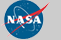Curator: David M. Giles NASA Official: Brent N. Holben Generated: 09/12/2019